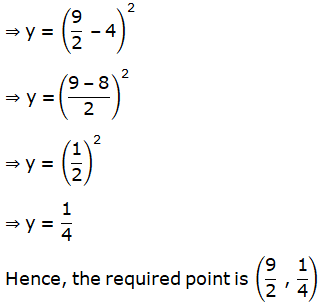# RD Sharma Solutions For Class 12 Maths Exercise 15.2 Chapter 15 Mean Value Theorems

Exercise 15.2 of Chapter 15 consists of problems based on verification of Lagrange’s theorem. Students who are unable to solve exercise-wise problems as per RD Sharma textbook can make use of solutions designed by expert faculty at BYJU’S. These solutions are described in the best possible way to provide a clear idea about the concepts to students. The PDF of RD Sharma Solutions for Class 12 Maths Chapter 15 Mean Value Theorem Exercise 15.2 is provided here. This PDF can be easily downloaded by the students. Some of the important topics of this exercise are listed below.

• Lagrange’s mean value theorem
• Geometrical interpretation of Lagrange’s mean value theorem
• Verification of Lagrange’s mean value theorem
• Proving inequalities by using Lagrange’s mean value theorem
• Miscellaneous applications of Lagrange’s mean value theorem

## RD Sharma Solutions For Class 12 Mean Value Theorems Exercise 15.2: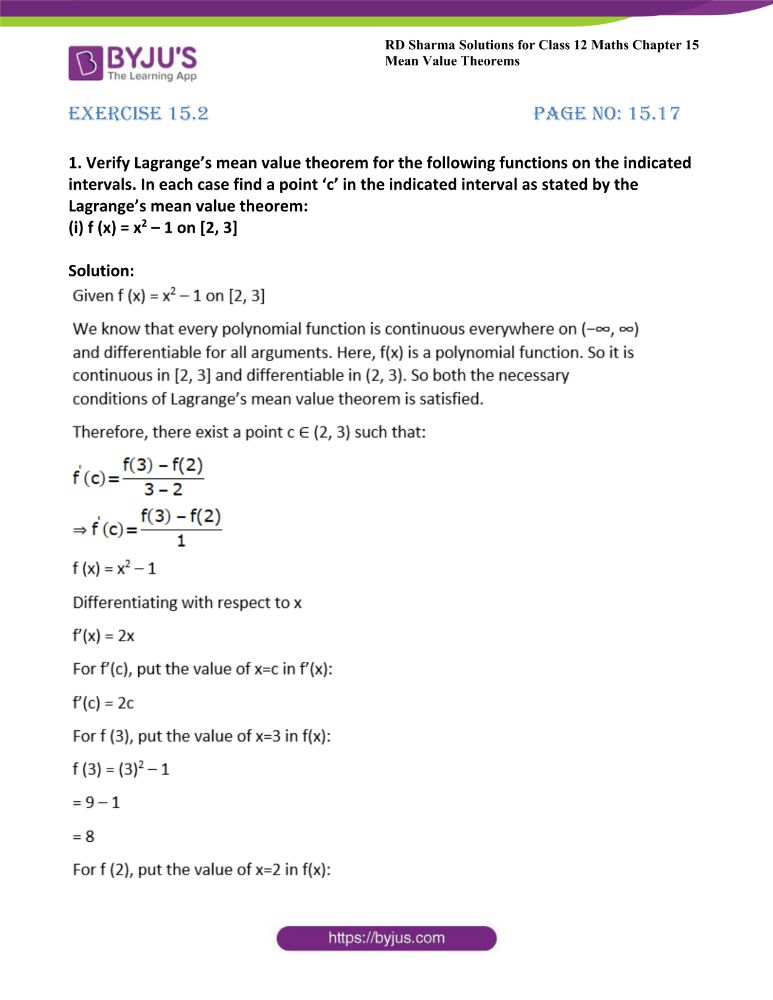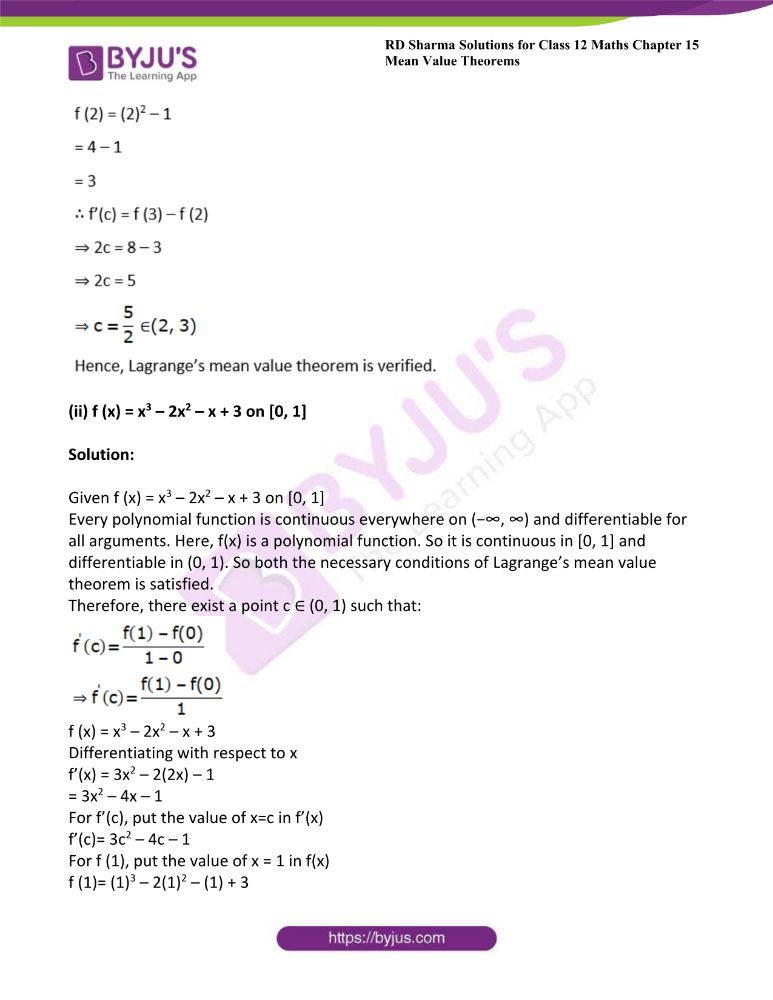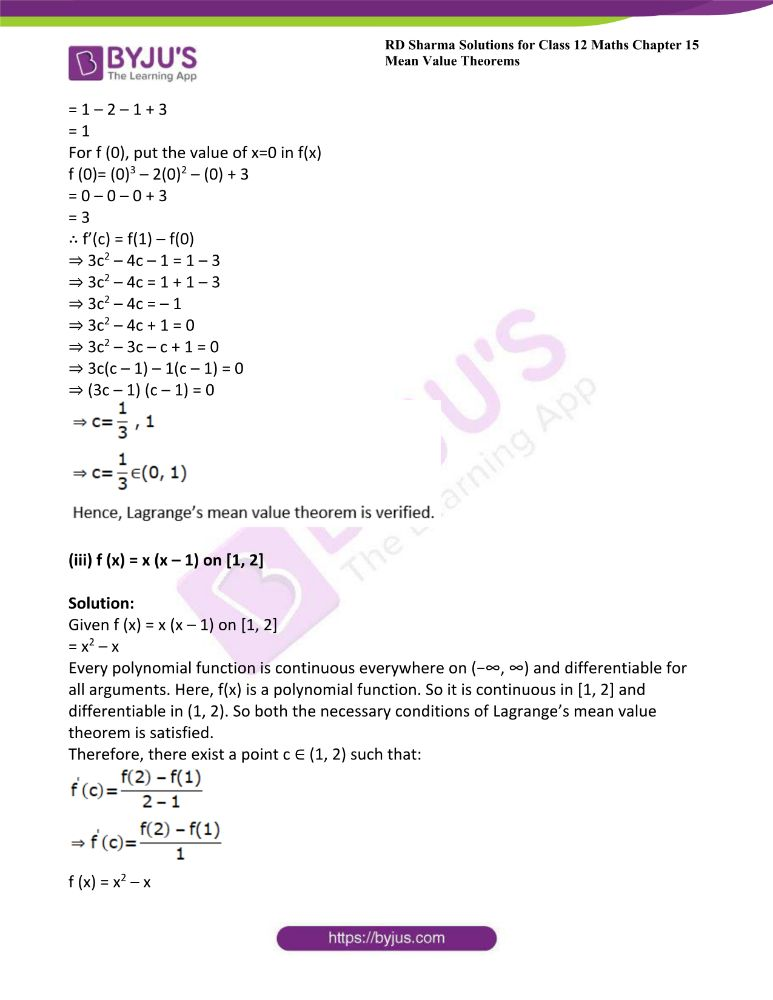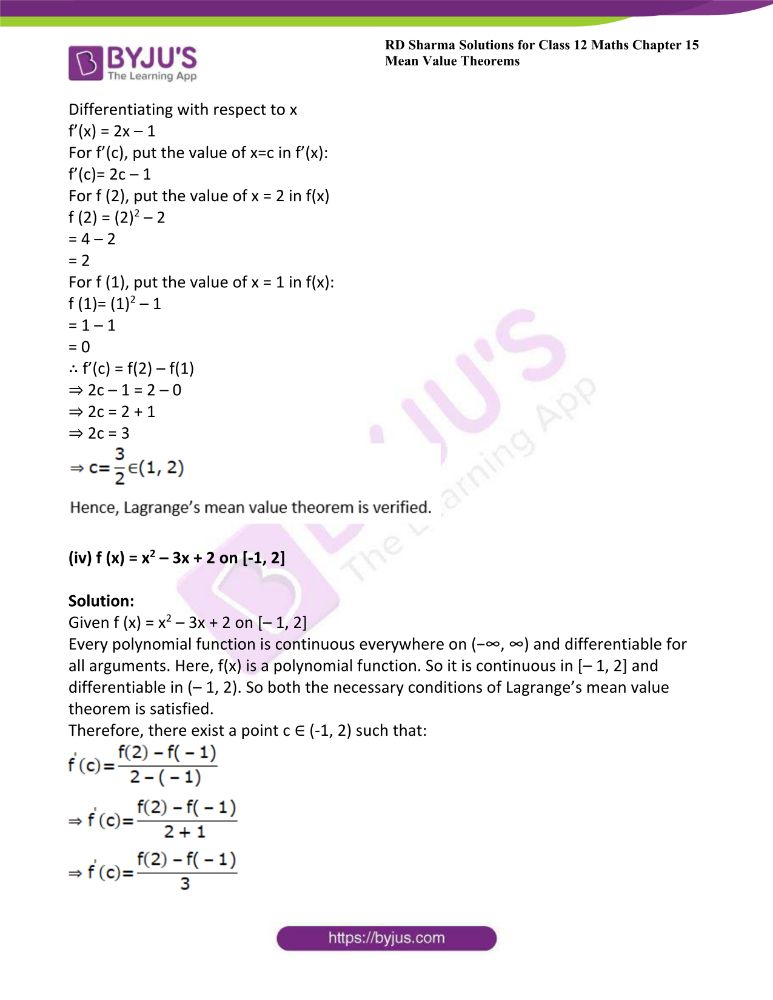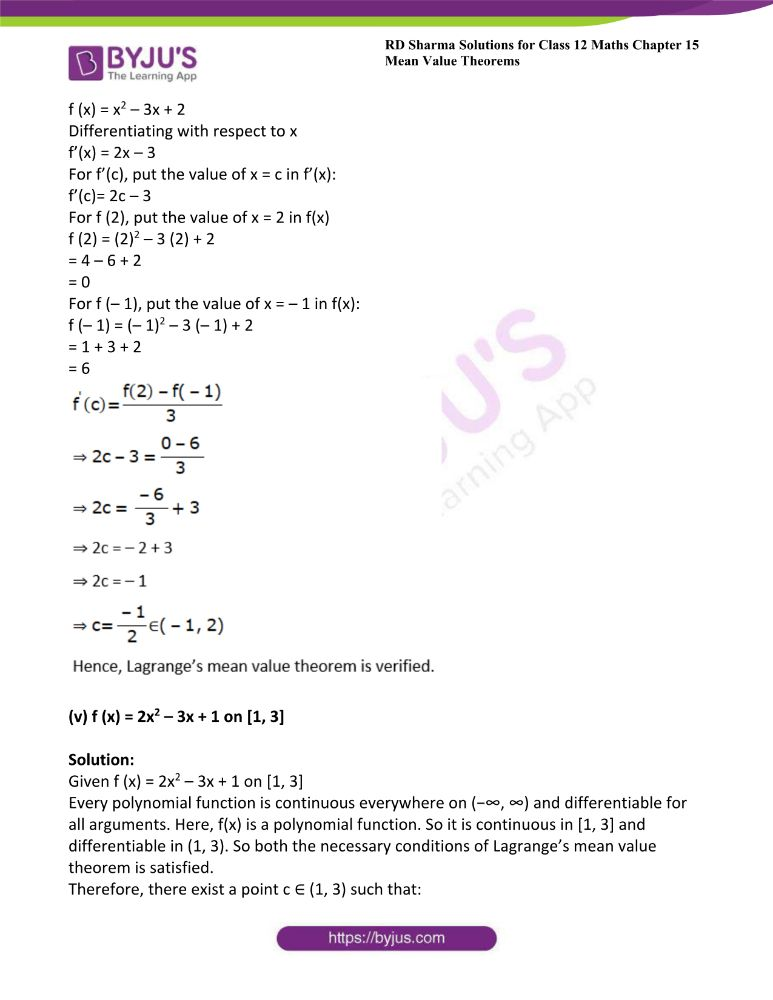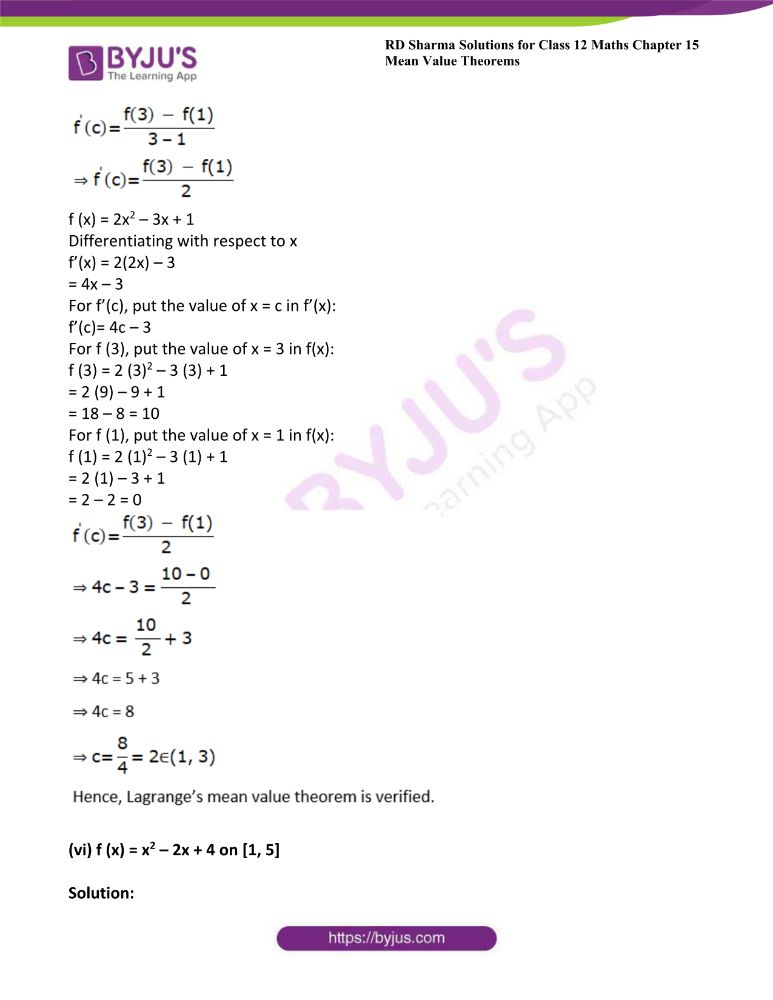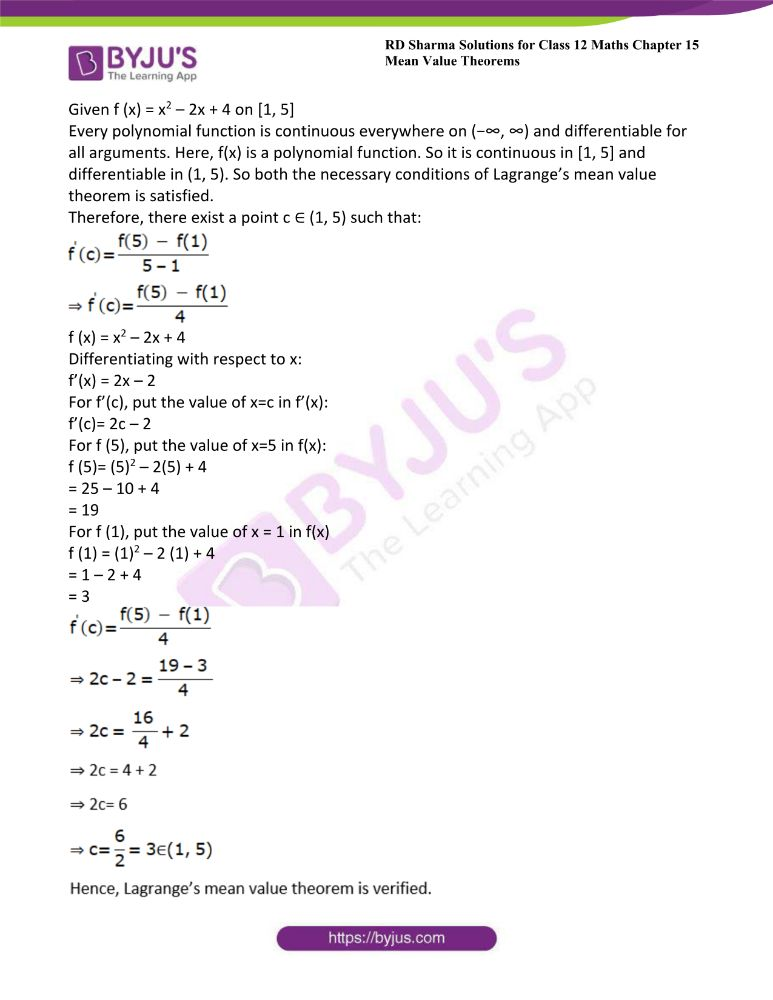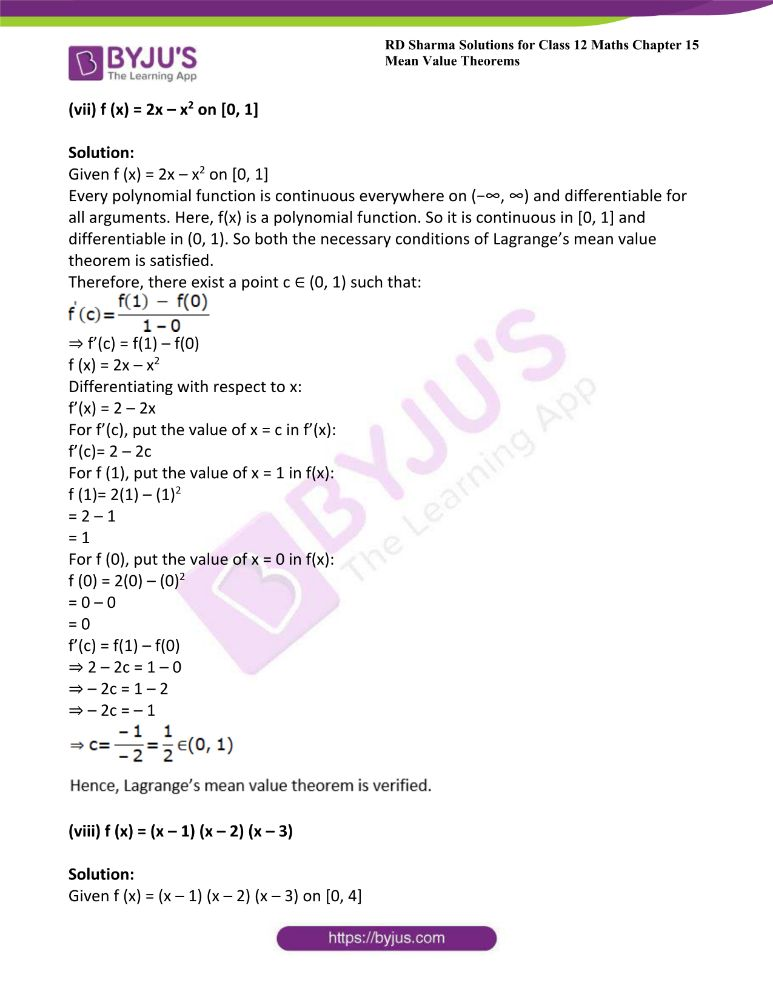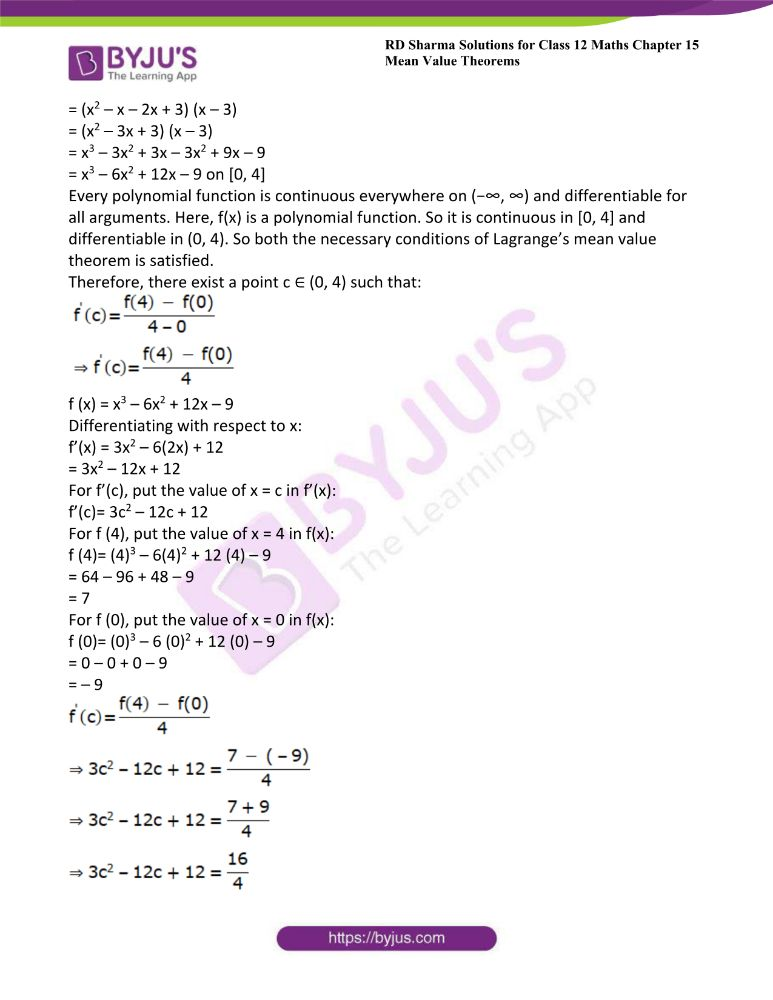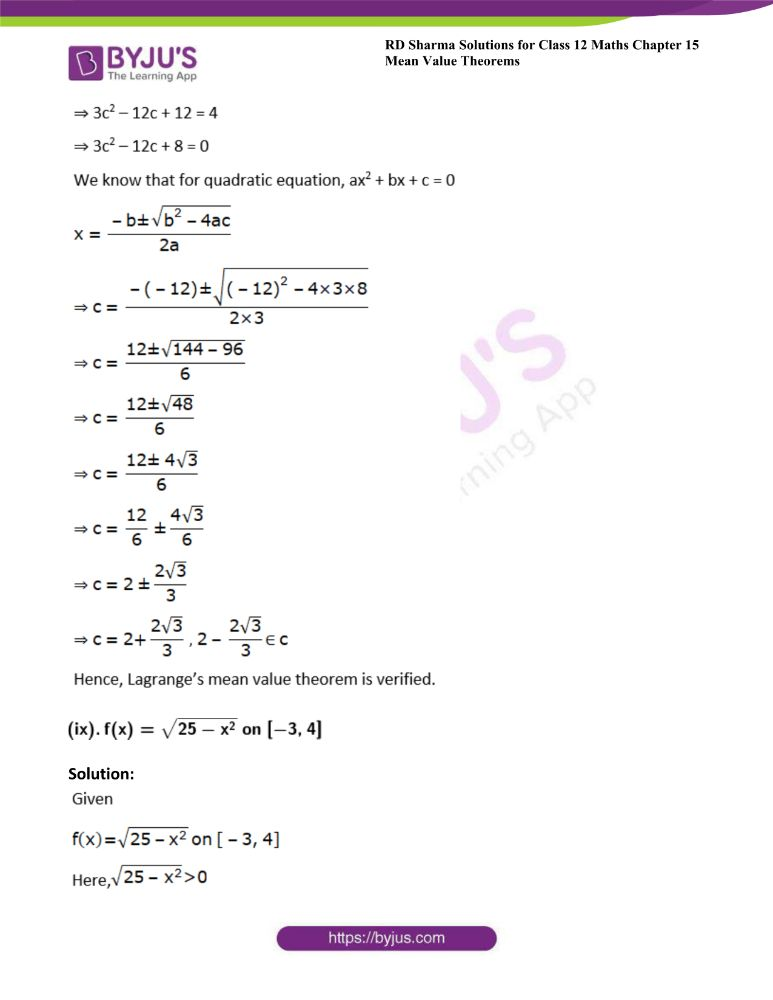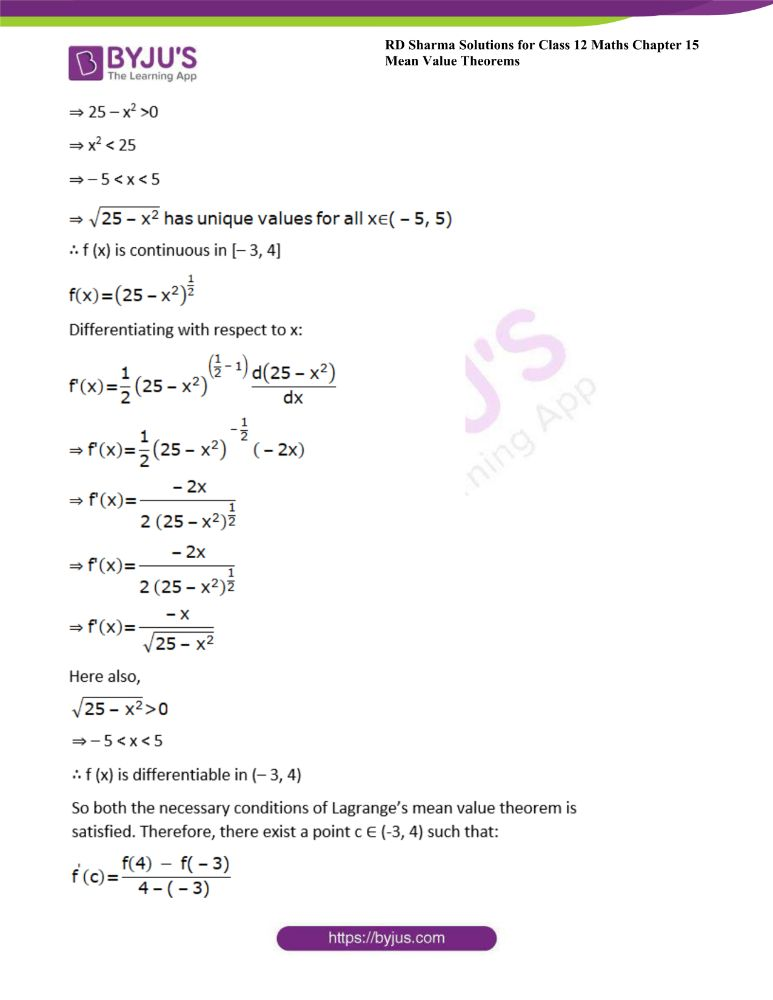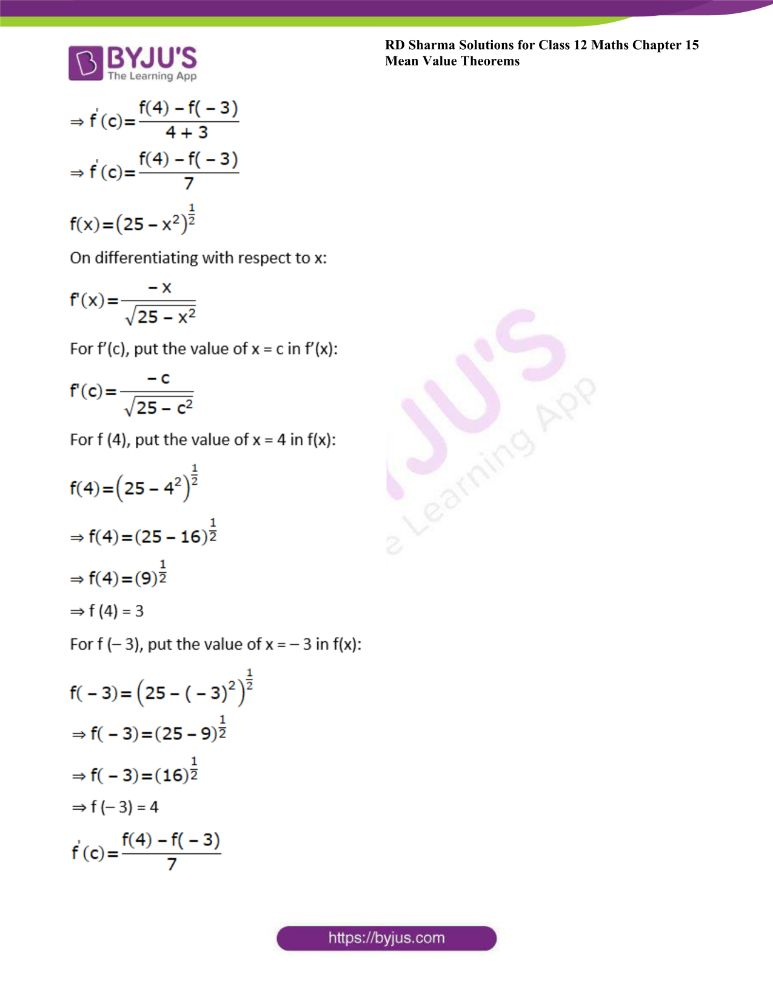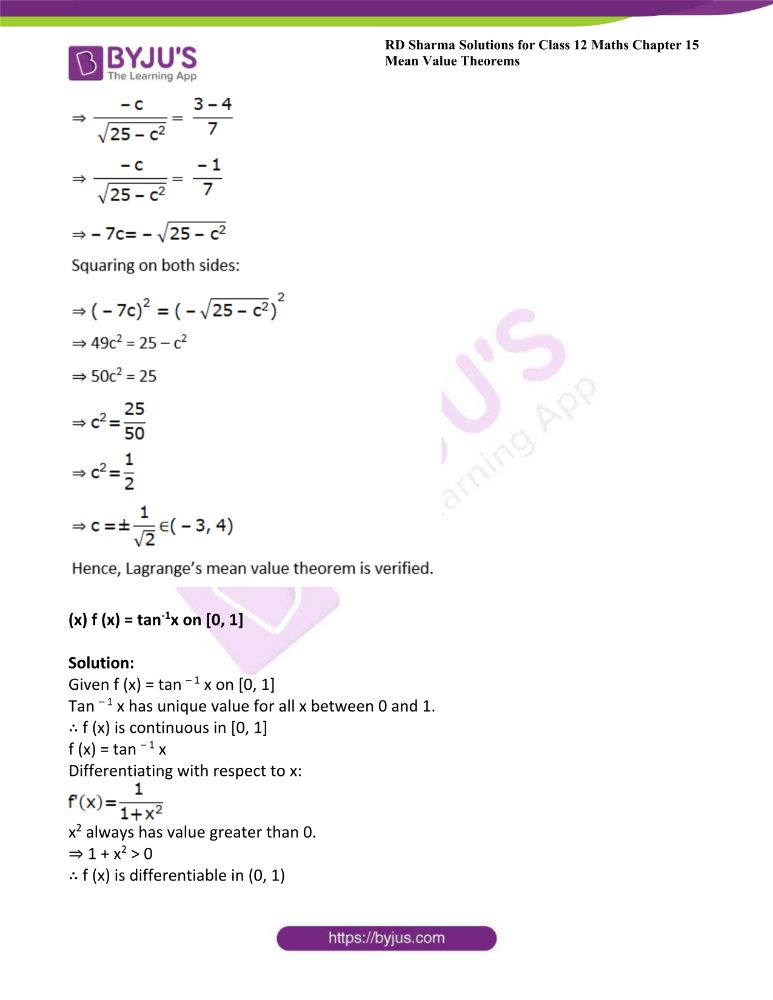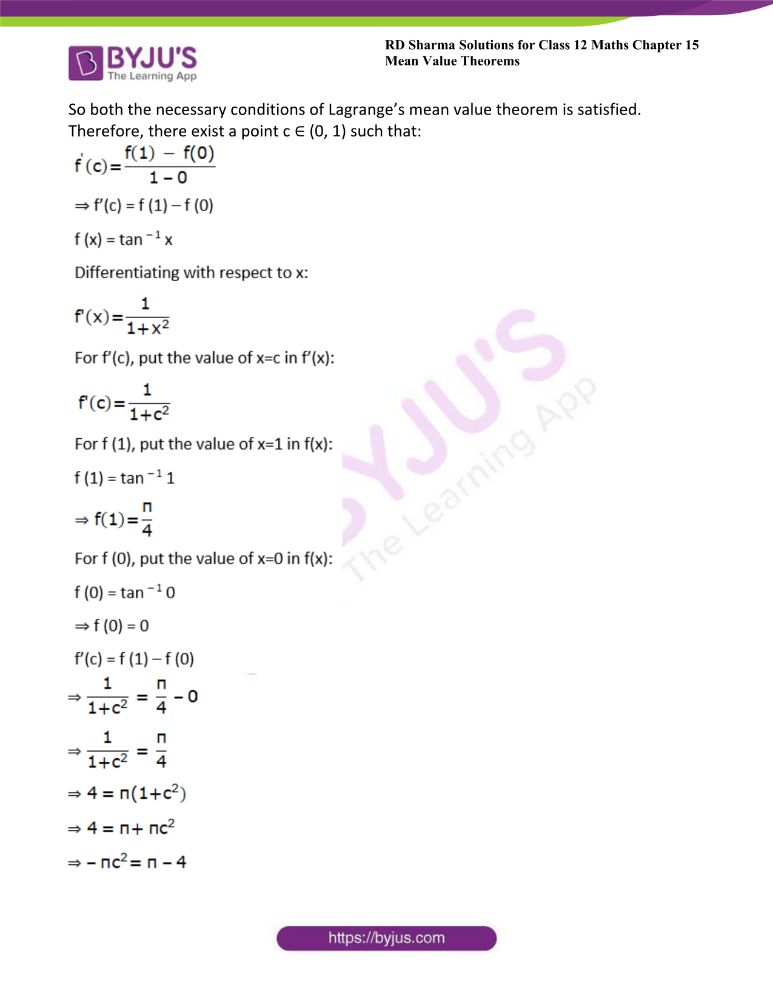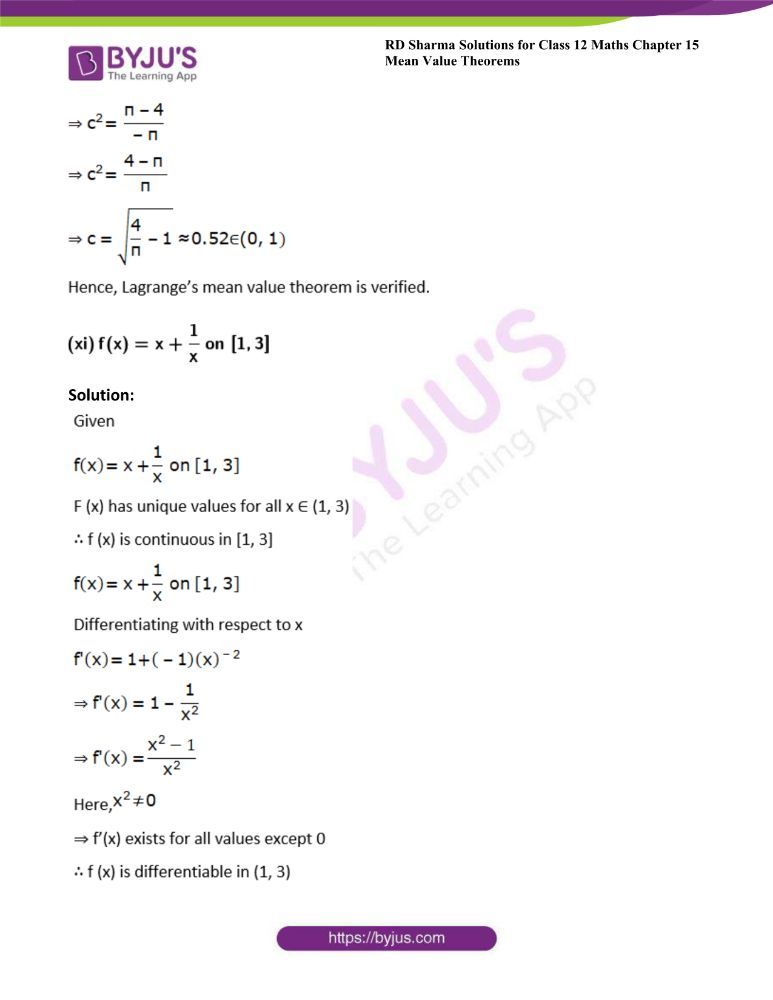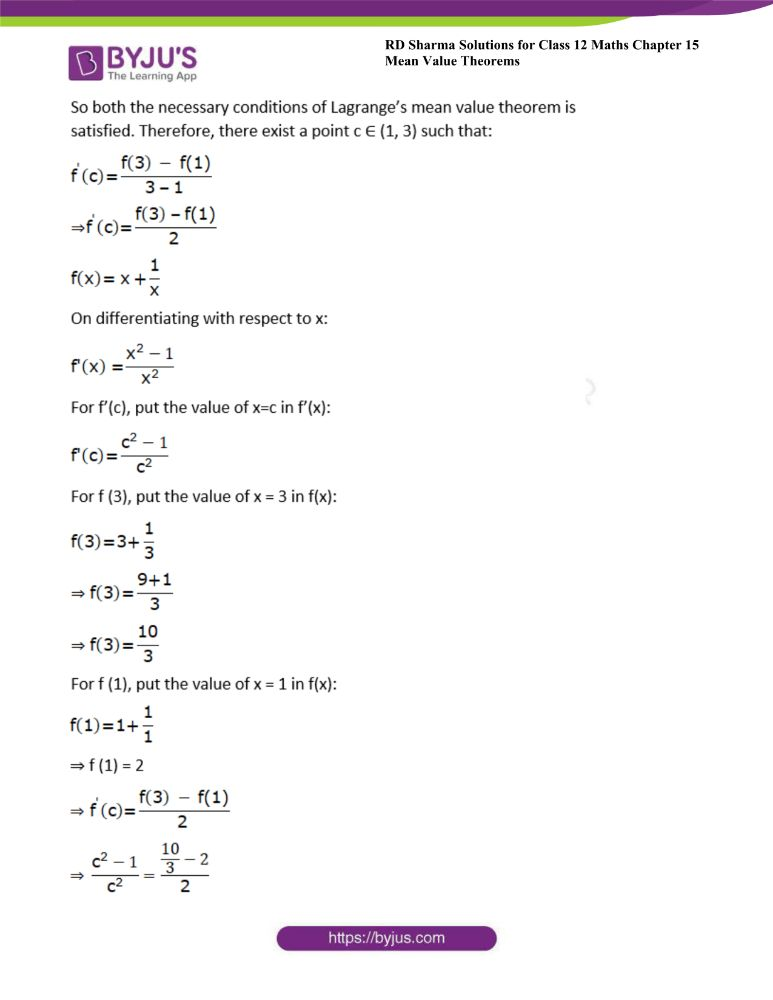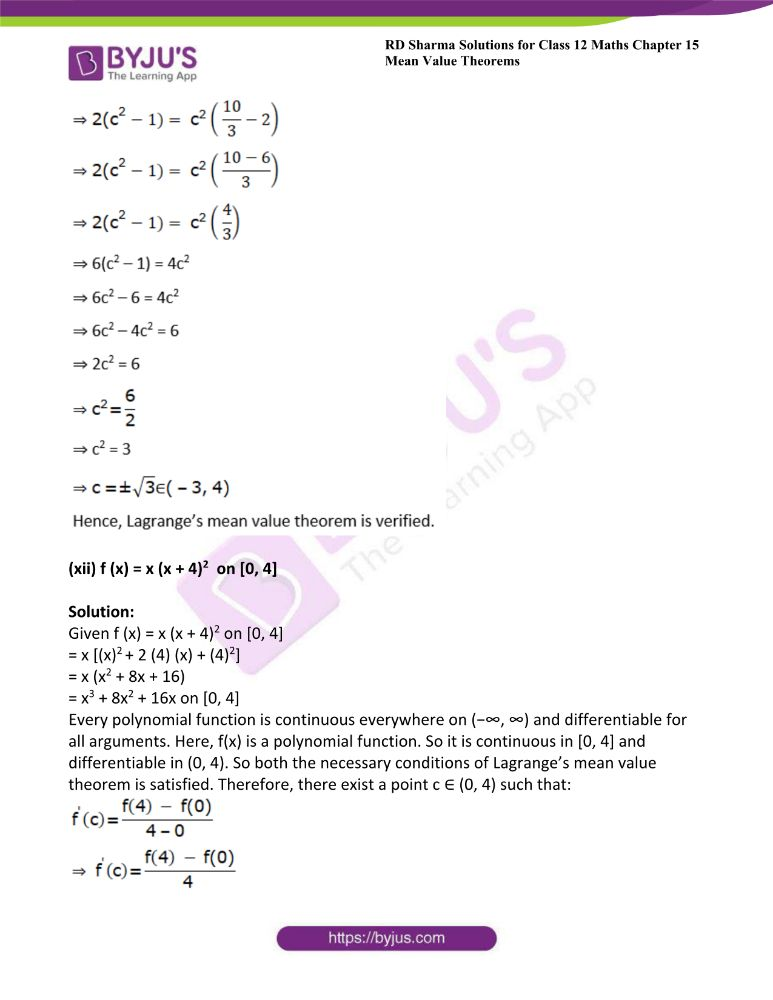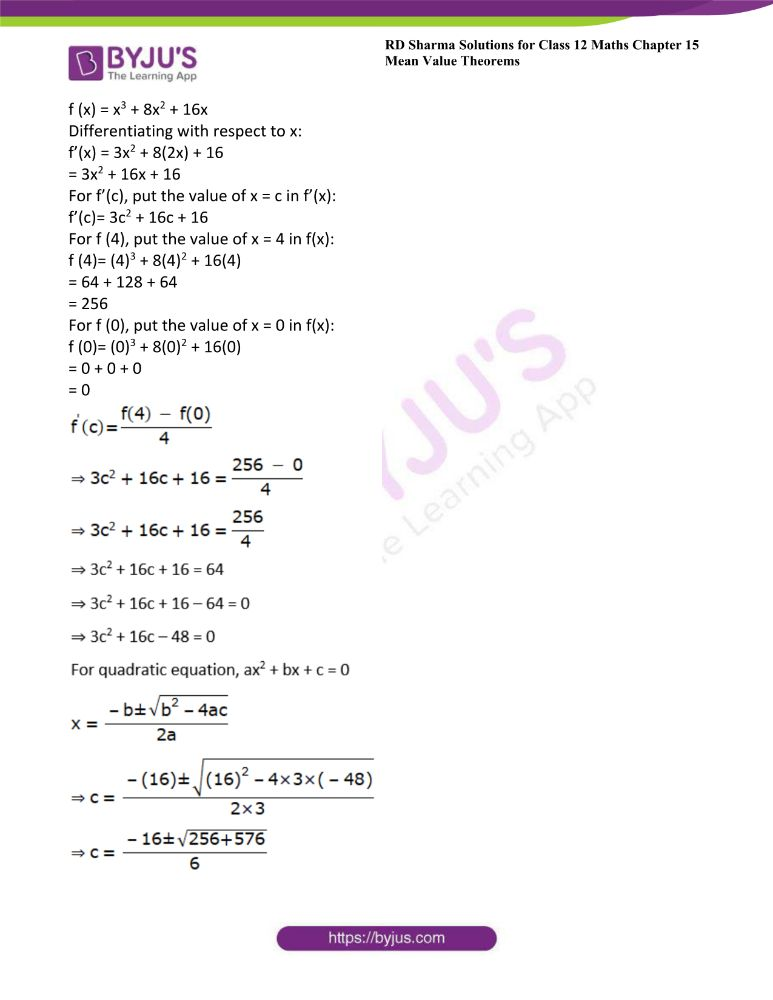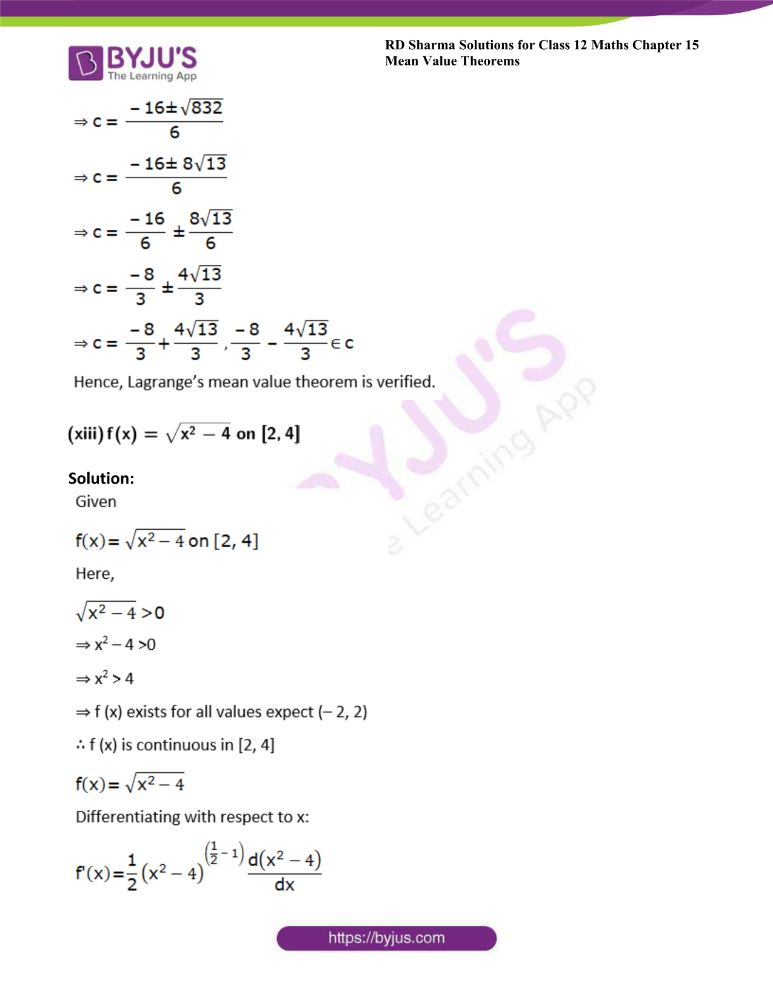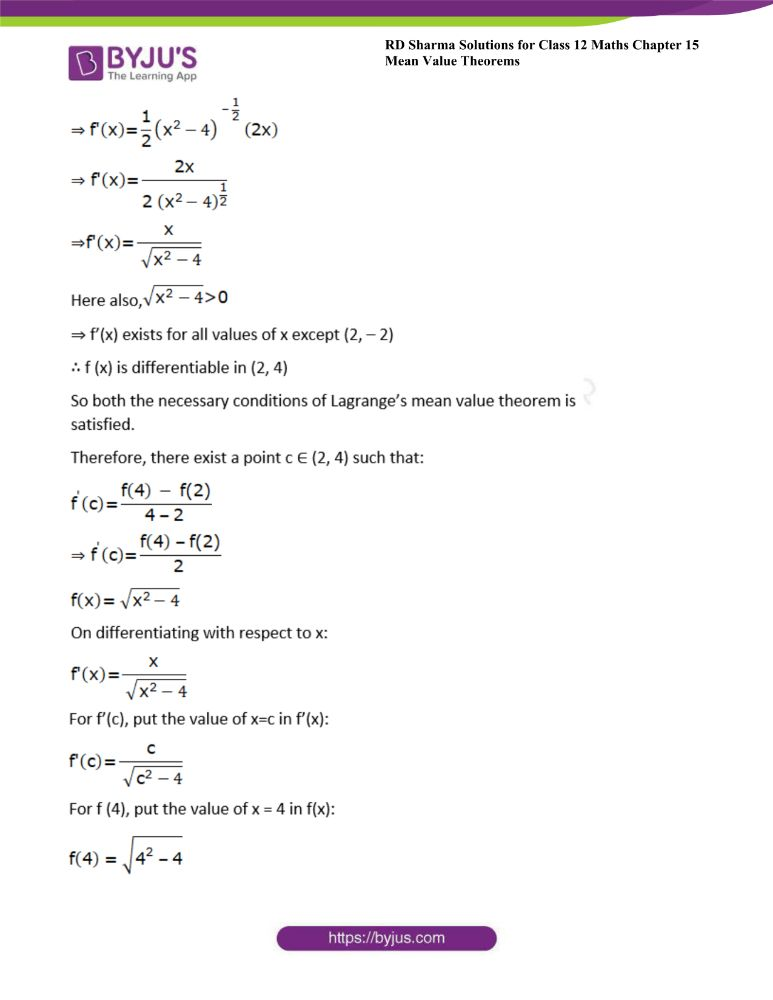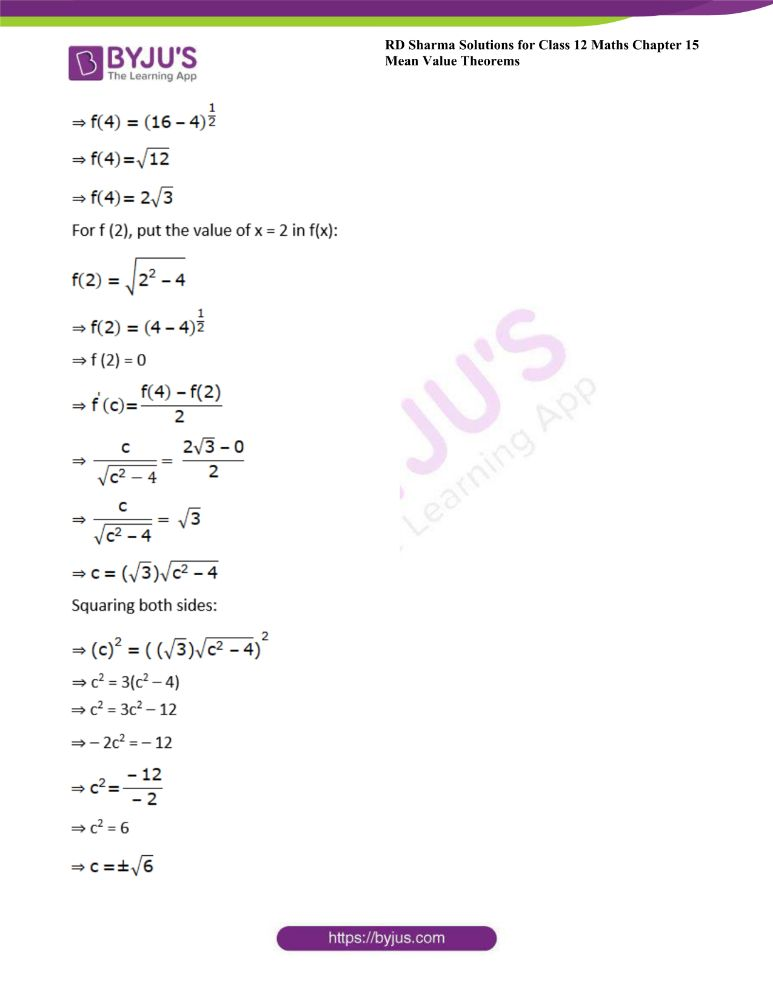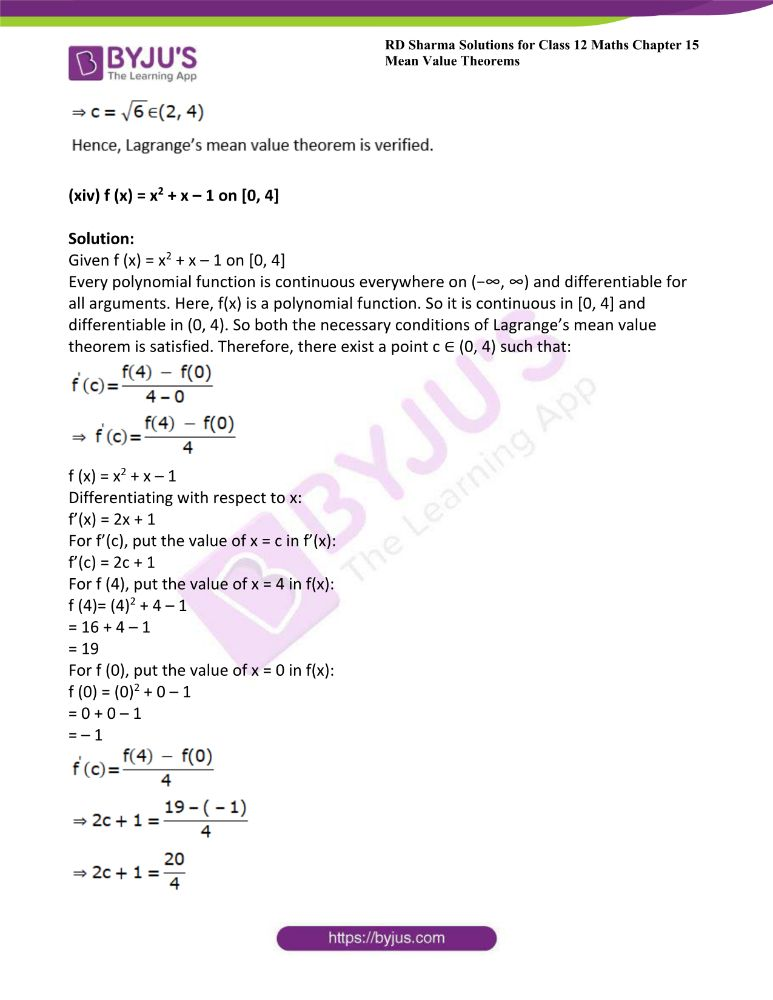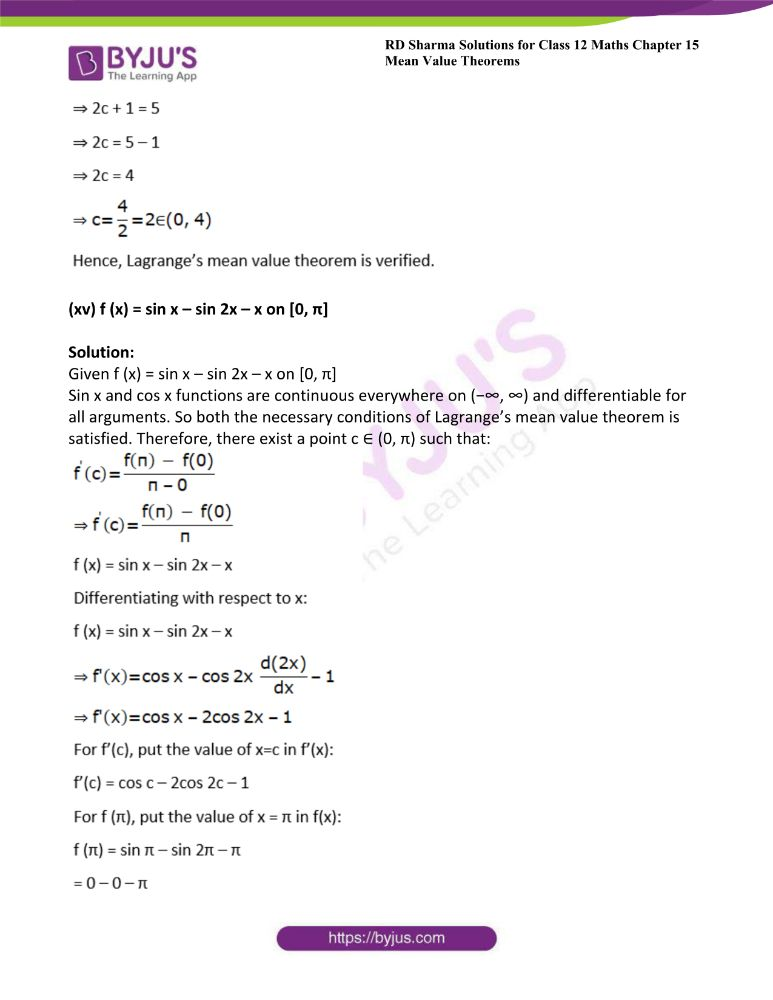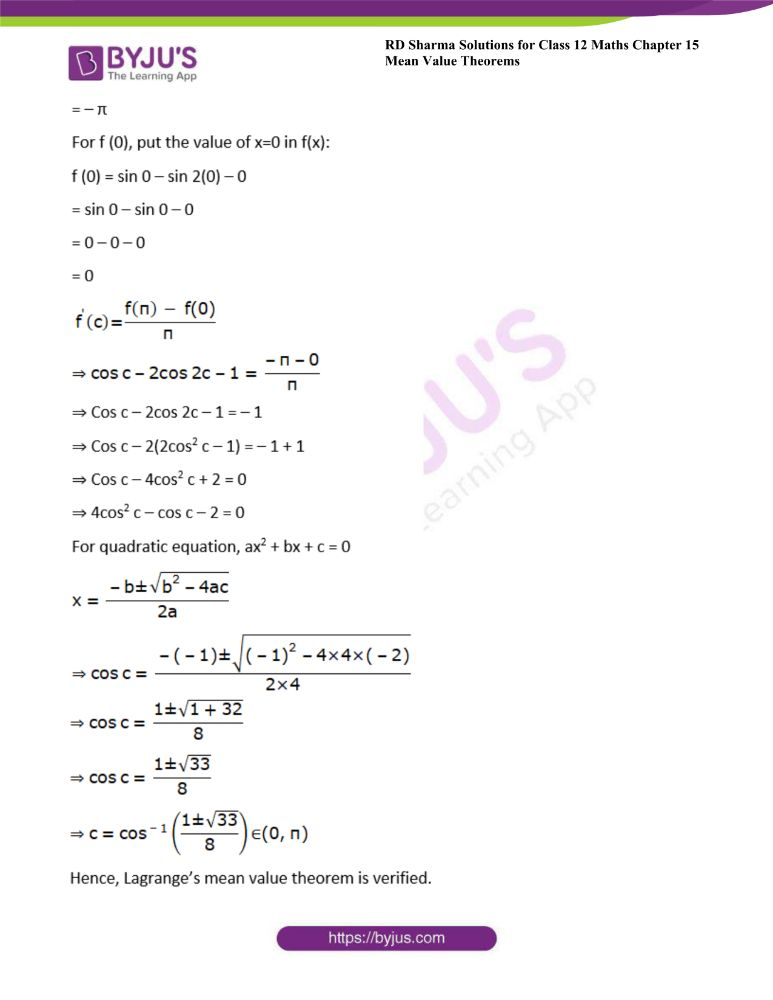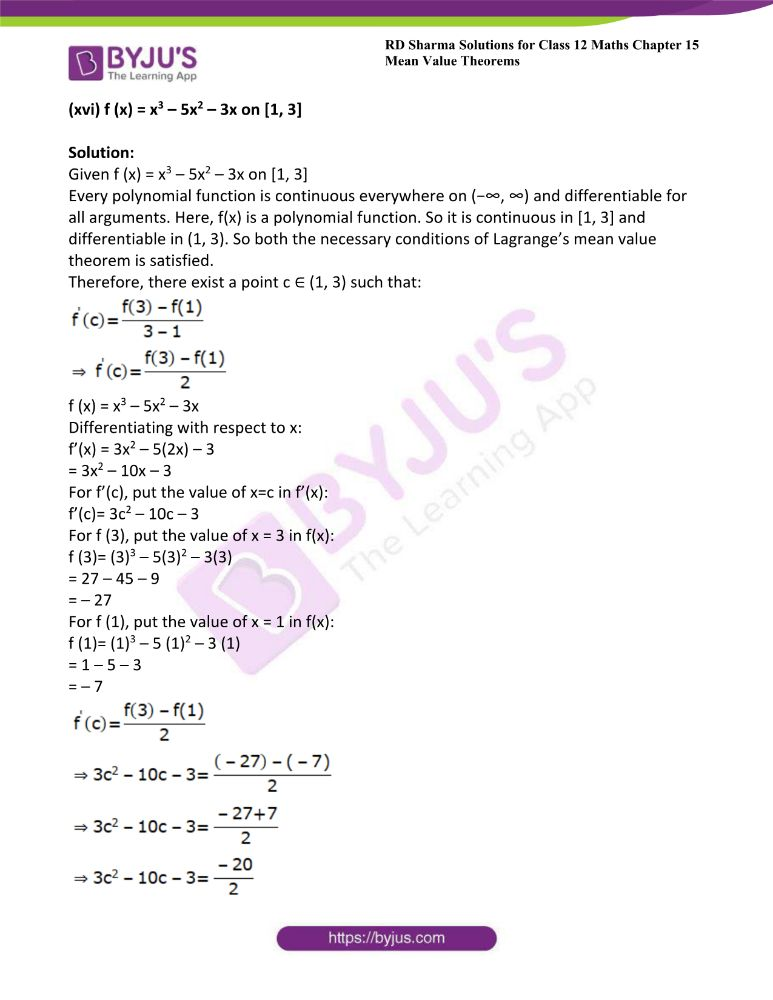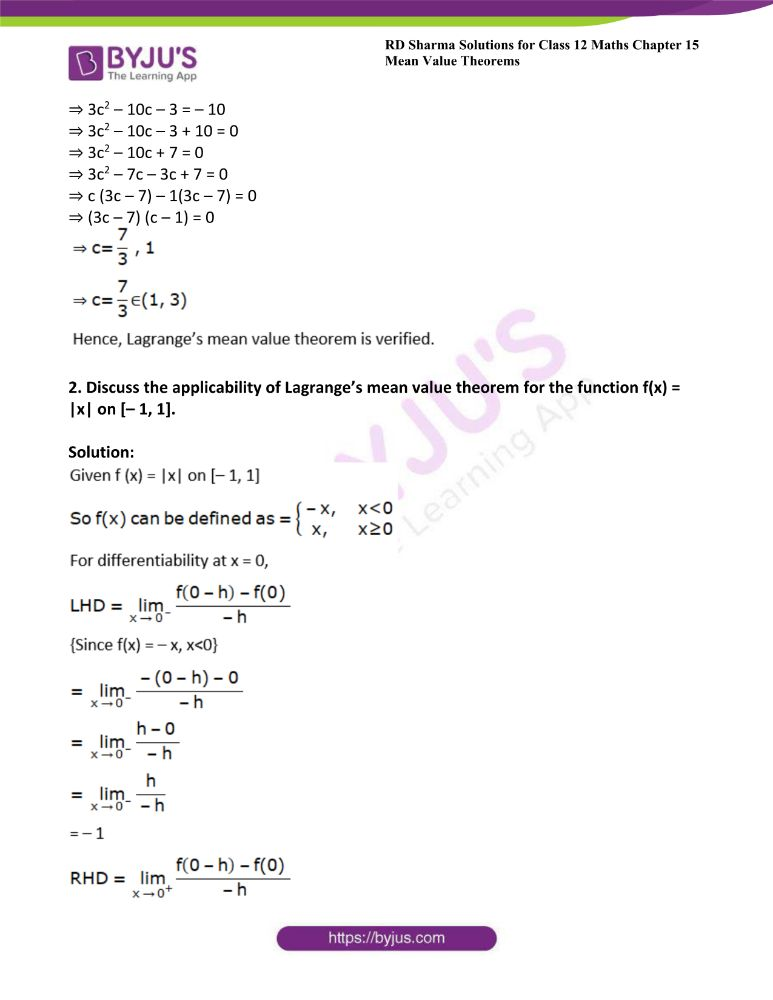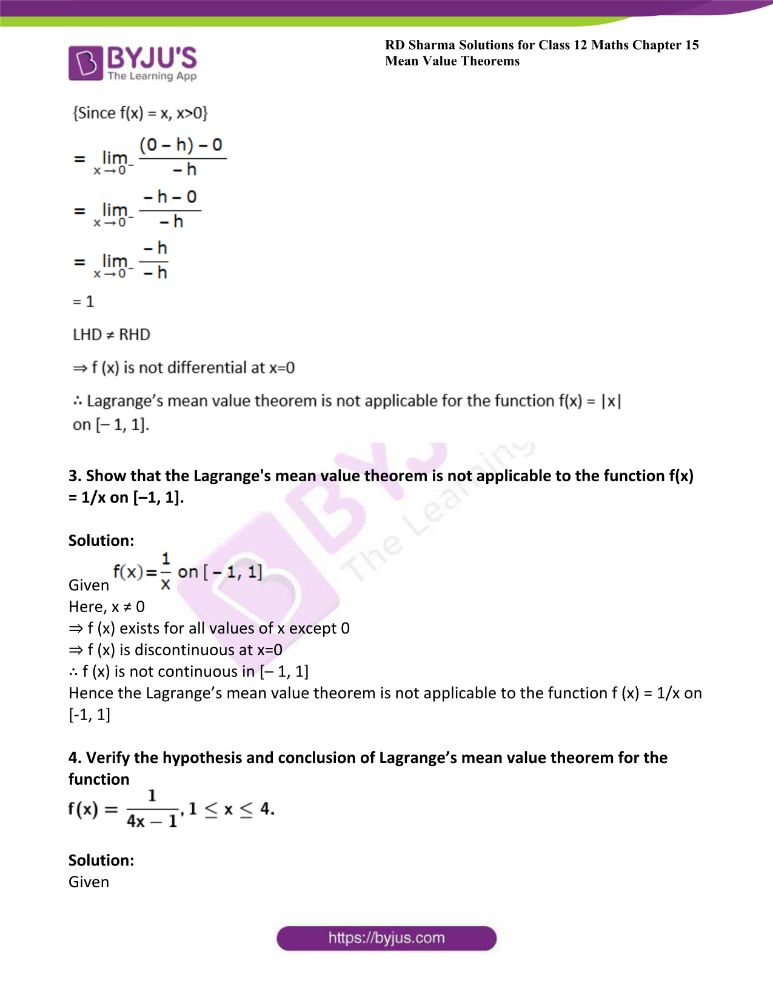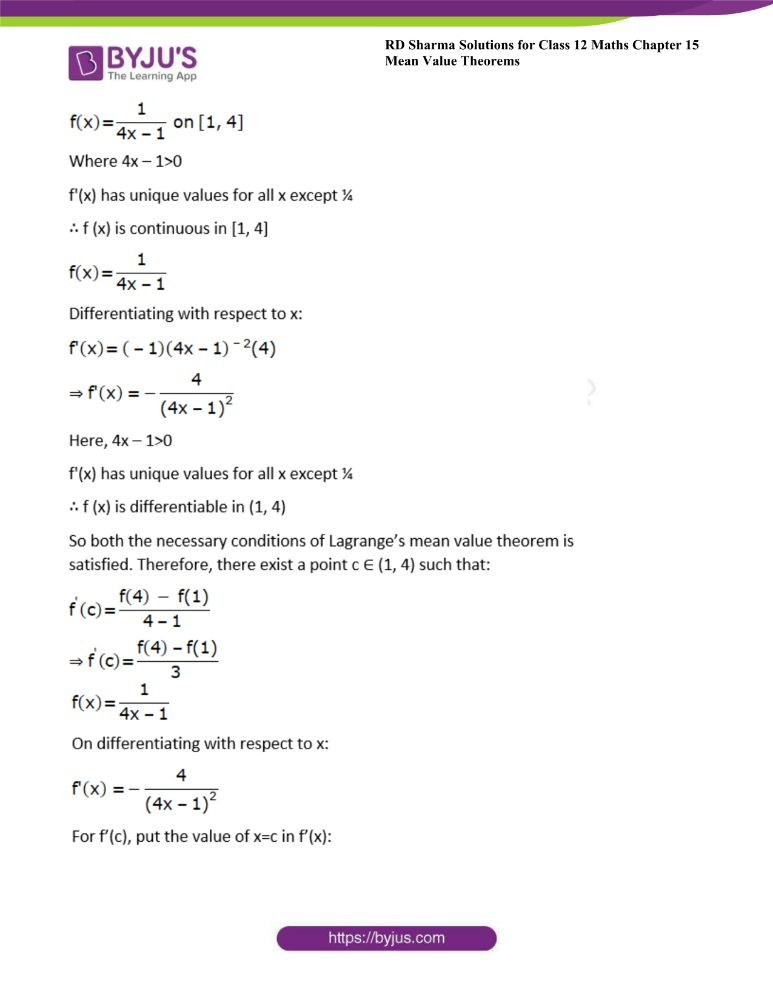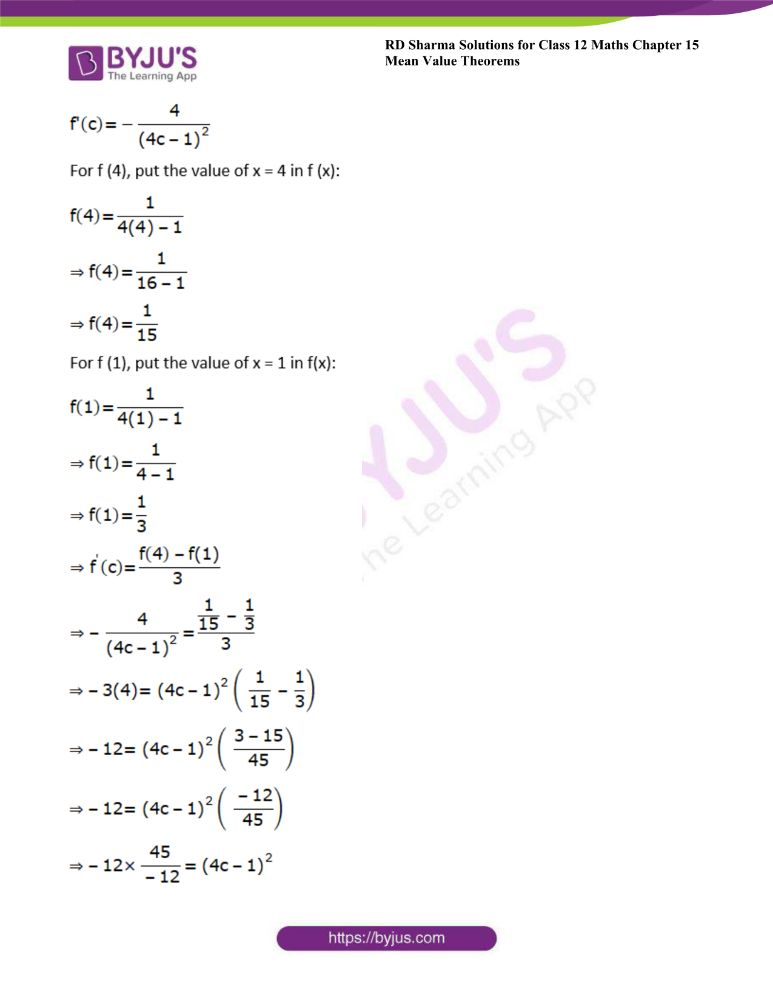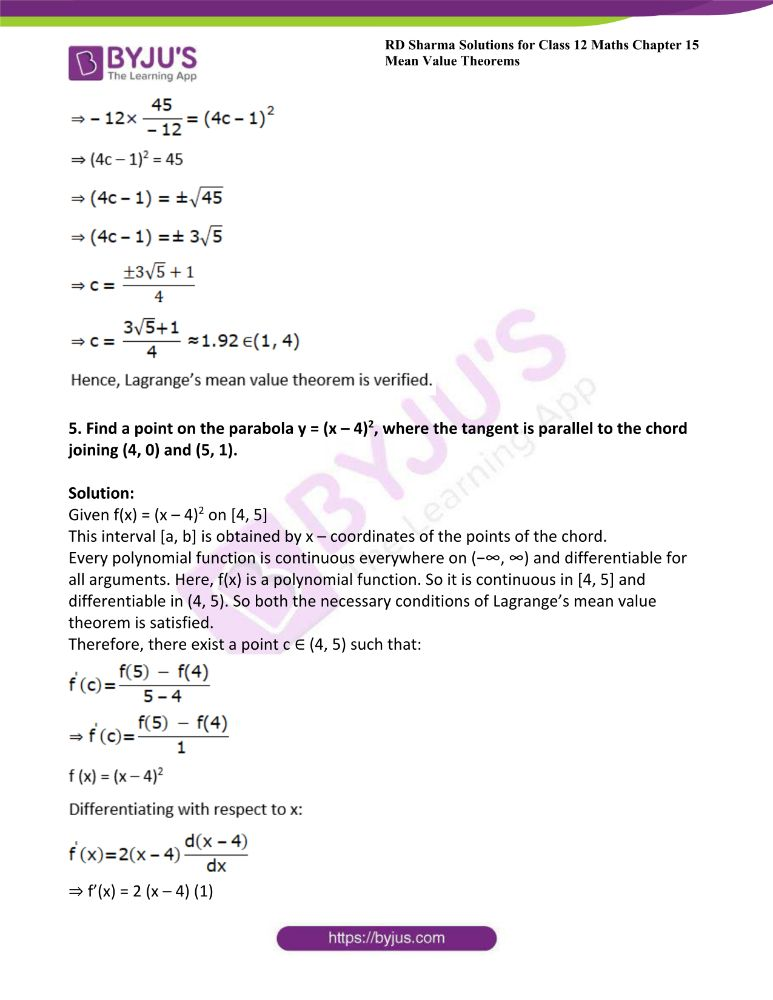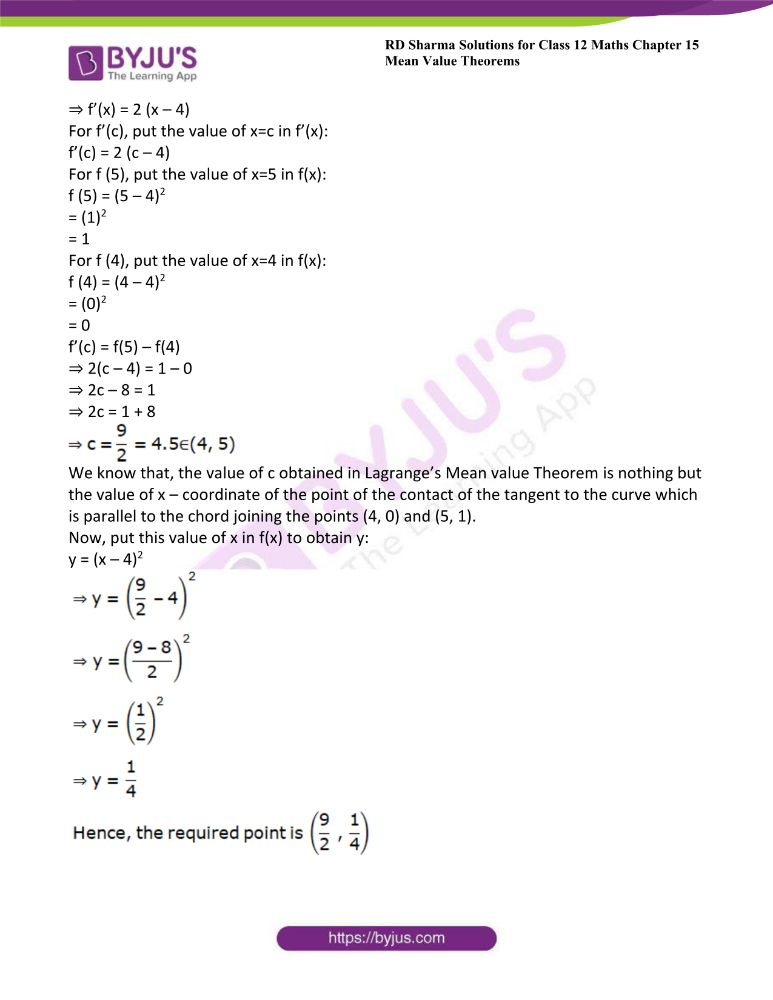### Access another exercise of RD Sharma Solutions For Class 12 Chapter 15 – Mean Value Theorems

Exercise 15.1 Solutions

### Access answers to Maths RD Sharma Solutions For Class 12 Chapter 15 – Mean Value Theorems Exercise 15.2

Page No: 15.17

1. Verify Lagrange’s mean value theorem for the following functions on the indicated intervals. In each case find a point ‘c’ in the indicated interval as stated by the Lagrange’s mean value theorem:

(i) f (x) = x2 – 1 on [2, 3]

Solution: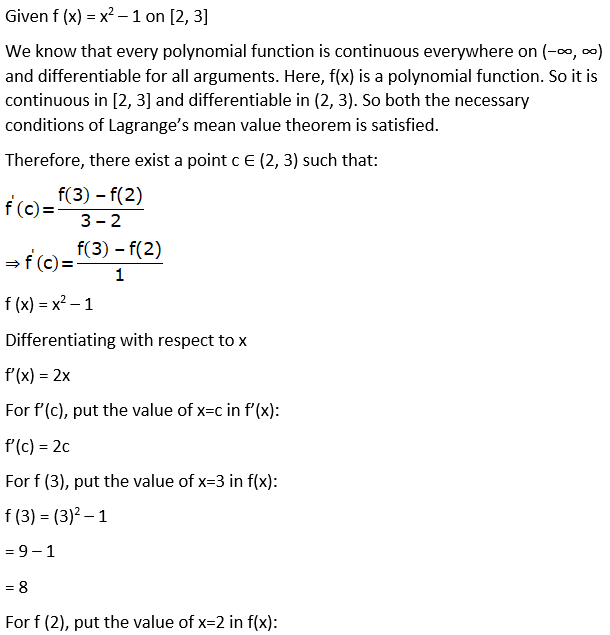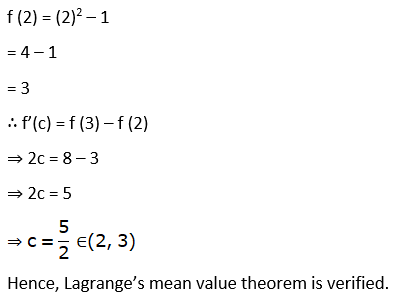(ii) f (x) = x3 – 2x2 – x + 3 on [0, 1]

Solution:

Given f (x) = x3 – 2x2 – x + 3 on [0, 1]

Every polynomial function is continuous everywhere on (−∞, ∞) and differentiable for all arguments. Here, f(x) is a polynomial function. So it is continuous in [0, 1] and differentiable in (0, 1). So both the necessary conditions of Lagrange’s mean value theorem is satisfied.

Therefore, there exist a point c ∈ (0, 1) such that: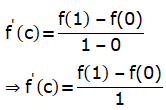f (x) = x3 – 2x2 – x + 3

Differentiating with respect to x

f’(x) = 3x2 – 2(2x) – 1

= 3x2 – 4x – 1

For f’(c), put the value of x=c in f’(x)

f’(c)= 3c2 – 4c – 1

For f (1), put the value of x = 1 in f(x)

f (1)= (1)3 – 2(1)2 – (1) + 3

= 1 – 2 – 1 + 3

= 1

For f (0), put the value of x=0 in f(x)

f (0)= (0)3 – 2(0)2 – (0) + 3

= 0 – 0 – 0 + 3

= 3

∴ f’(c) = f(1) – f(0)

⇒ 3c2 – 4c – 1 = 1 – 3

⇒ 3c2 – 4c = 1 + 1 – 3

⇒ 3c2 – 4c = – 1

⇒ 3c2 – 4c + 1 = 0

⇒ 3c2 – 3c – c + 1 = 0

⇒ 3c(c – 1) – 1(c – 1) = 0

⇒ (3c – 1) (c – 1) = 0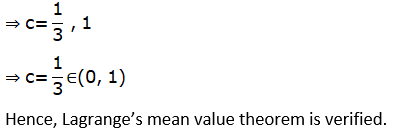(iii) f (x) = x (x – 1) on [1, 2]

Solution:

Given f (x) = x (x – 1) on [1, 2]

= x2 – x

Every polynomial function is continuous everywhere on (−∞, ∞) and differentiable for all arguments. Here, f(x) is a polynomial function. So it is continuous in [1, 2] and differentiable in (1, 2). So both the necessary conditions of Lagrange’s mean value theorem is satisfied.

Therefore, there exist a point c ∈ (1, 2) such that: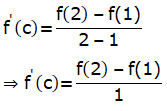f (x) = x2 – x

Differentiating with respect to x

f’(x) = 2x – 1

For f’(c), put the value of x=c in f’(x):

f’(c)= 2c – 1

For f (2), put the value of x = 2 in f(x)

f (2) = (2)2 – 2

= 4 – 2

= 2

For f (1), put the value of x = 1 in f(x):

f (1)= (1)2 – 1

= 1 – 1

= 0

∴ f’(c) = f(2) – f(1)

⇒ 2c – 1 = 2 – 0

⇒ 2c = 2 + 1

⇒ 2c = 3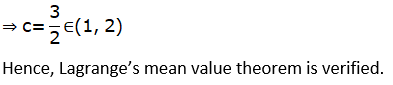(iv) f (x) = x2 – 3x + 2 on [-1, 2]

Solution:

Given f (x) = x2 – 3x + 2 on [– 1, 2]

Every polynomial function is continuous everywhere on (−∞, ∞) and differentiable for all arguments. Here, f(x) is a polynomial function. So it is continuous in [– 1, 2] and differentiable in (– 1, 2). So both the necessary conditions of Lagrange’s mean value theorem is satisfied.

Therefore, there exist a point c ∈ (-1, 2) such that: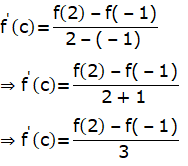f (x) = x2 – 3x + 2

Differentiating with respect to x

f’(x) = 2x – 3

For f’(c), put the value of x = c in f’(x):

f’(c)= 2c – 3

For f (2), put the value of x = 2 in f(x)

f (2) = (2)2 – 3 (2) + 2

= 4 – 6 + 2

= 0

For f (– 1), put the value of x = – 1 in f(x):

f (– 1) = (– 1)2 – 3 (– 1) + 2

= 1 + 3 + 2

= 6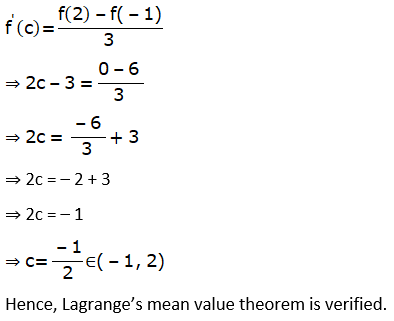(v) f (x) = 2x2 – 3x + 1 on [1, 3]

Solution:

Given f (x) = 2x2 – 3x + 1 on [1, 3]

Every polynomial function is continuous everywhere on (−∞, ∞) and differentiable for all arguments. Here, f(x) is a polynomial function. So it is continuous in [1, 3] and differentiable in (1, 3). So both the necessary conditions of Lagrange’s mean value theorem is satisfied.

Therefore, there exist a point c ∈ (1, 3) such that: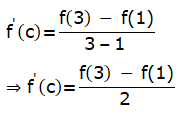f (x) = 2x2 – 3x + 1

Differentiating with respect to x

f’(x) = 2(2x) – 3

= 4x – 3

For f’(c), put the value of x = c in f’(x):

f’(c)= 4c – 3

For f (3), put the value of x = 3 in f(x):

f (3) = 2 (3)2 – 3 (3) + 1

= 2 (9) – 9 + 1

= 18 – 8 = 10

For f (1), put the value of x = 1 in f(x):

f (1) = 2 (1)2 – 3 (1) + 1

= 2 (1) – 3 + 1

= 2 – 2 = 0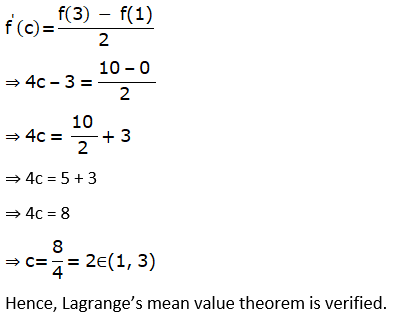(vi) f (x) = x2 – 2x + 4 on [1, 5]

Solution:

Given f (x) = x2 – 2x + 4 on [1, 5]

Every polynomial function is continuous everywhere on (−∞, ∞) and differentiable for all arguments. Here, f(x) is a polynomial function. So it is continuous in [1, 5] and differentiable in (1, 5). So both the necessary conditions of Lagrange’s mean value theorem is satisfied.

Therefore, there exist a point c ∈ (1, 5) such that:f (x) = x2 – 2x + 4

Differentiating with respect to x:

f’(x) = 2x – 2

For f’(c), put the value of x=c in f’(x):

f’(c)= 2c – 2

For f (5), put the value of x=5 in f(x):

f (5)= (5)2 – 2(5) + 4

= 25 – 10 + 4

= 19

For f (1), put the value of x = 1 in f(x)

f (1) = (1)2 – 2 (1) + 4

= 1 – 2 + 4

= 3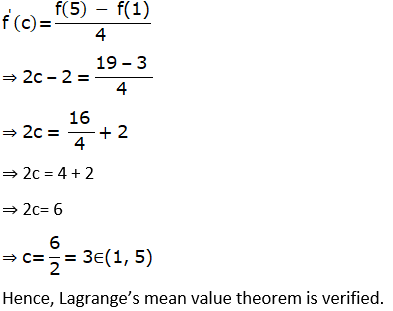(vii) f (x) = 2x – x2 on [0, 1]

Solution:

Given f (x) = 2x – x2 on [0, 1]

Every polynomial function is continuous everywhere on (−∞, ∞) and differentiable for all arguments. Here, f(x) is a polynomial function. So it is continuous in [0, 1] and differentiable in (0, 1). So both the necessary conditions of Lagrange’s mean value theorem is satisfied.

Therefore, there exist a point c ∈ (0, 1) such that: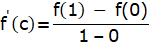⇒ f’(c) = f(1) – f(0)

f (x) = 2x – x2

Differentiating with respect to x:

f’(x) = 2 – 2x

For f’(c), put the value of x = c in f’(x):

f’(c)= 2 – 2c

For f (1), put the value of x = 1 in f(x):

f (1)= 2(1) – (1)2

= 2 – 1

= 1

For f (0), put the value of x = 0 in f(x):

f (0) = 2(0) – (0)2

= 0 – 0

= 0

f’(c) = f(1) – f(0)

⇒ 2 – 2c = 1 – 0

⇒ – 2c = 1 – 2

⇒ – 2c = – 1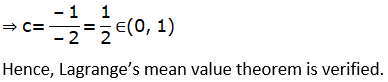(viii) f (x) = (x – 1) (x – 2) (x – 3)

Solution:

Given f (x) = (x – 1) (x – 2) (x – 3) on [0, 4]

= (x2 – x – 2x + 3) (x – 3)

= (x2 – 3x + 3) (x – 3)

= x3 – 3x2 + 3x – 3x2 + 9x – 9

= x3 – 6x2 + 12x – 9 on [0, 4]

Every polynomial function is continuous everywhere on (−∞, ∞) and differentiable for all arguments. Here, f(x) is a polynomial function. So it is continuous in [0, 4] and differentiable in (0, 4). So both the necessary conditions of Lagrange’s mean value theorem is satisfied.

Therefore, there exist a point c ∈ (0, 4) such that: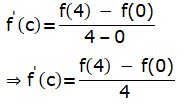f (x) = x3 – 6x2 + 12x – 9

Differentiating with respect to x:

f’(x) = 3x2 – 6(2x) + 12

= 3x2 – 12x + 12

For f’(c), put the value of x = c in f’(x):

f’(c)= 3c2 – 12c + 12

For f (4), put the value of x = 4 in f(x):

f (4)= (4)3 – 6(4)2 + 12 (4) – 9

= 64 – 96 + 48 – 9

= 7

For f (0), put the value of x = 0 in f(x):

f (0)= (0)3 – 6 (0)2 + 12 (0) – 9

= 0 – 0 + 0 – 9

= – 9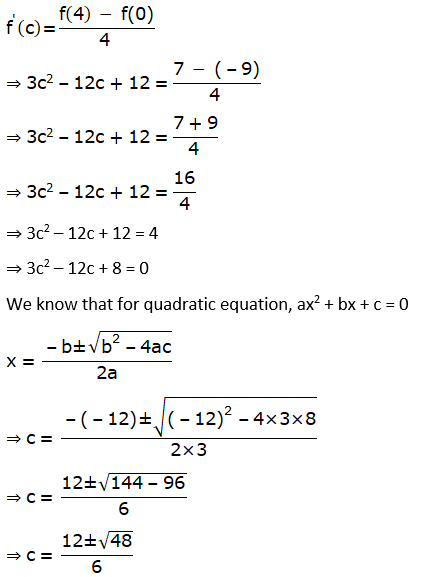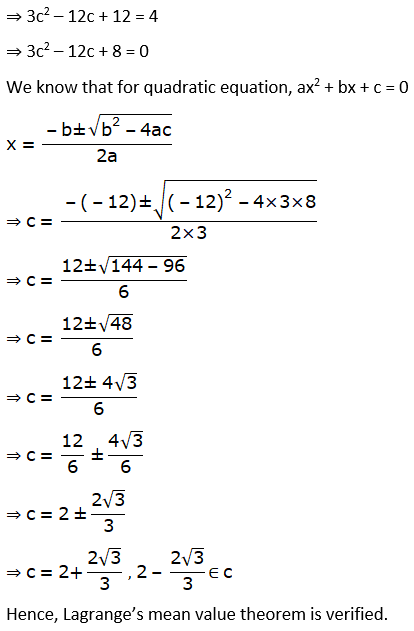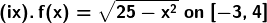Solution: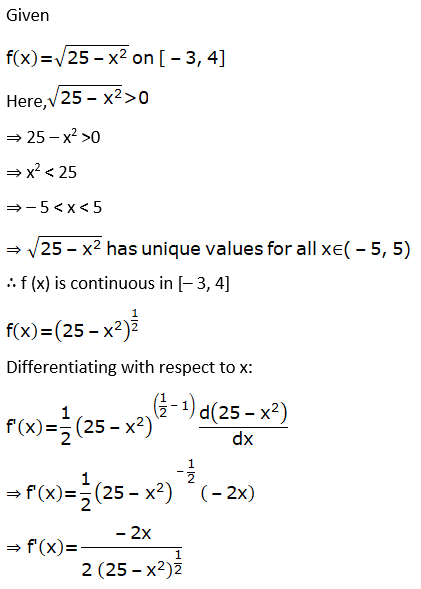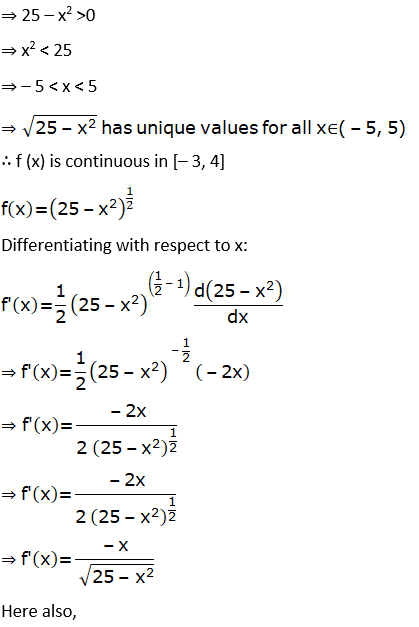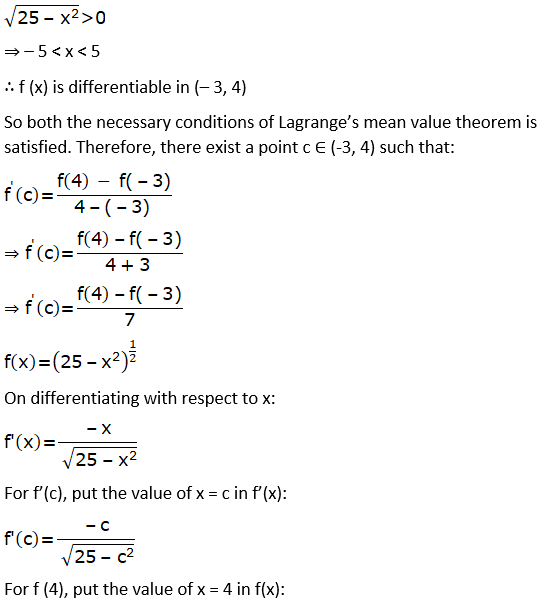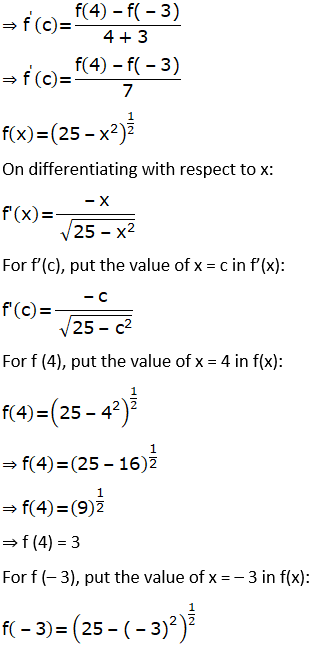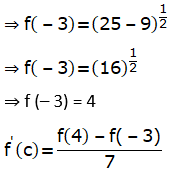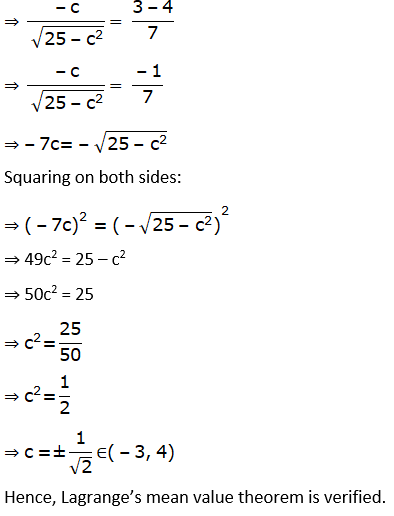(x) f (x) = tan-1x on [0, 1]

Solution:

Given f (x) = tan – 1 x on [0, 1]

Tan – 1 x has unique value for all x between 0 and 1.

∴ f (x) is continuous in [0, 1]

f (x) = tan – 1 x

Differentiating with respect to x: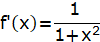x2 always has value greater than 0.

⇒ 1 + x2 > 0

∴ f (x) is differentiable in (0, 1)

So both the necessary conditions of Lagrange’s mean value theorem is satisfied. Therefore, there exist a point c ∈ (0, 1) such that: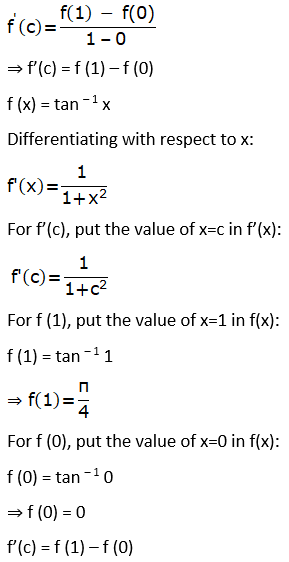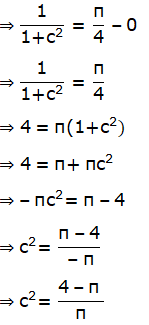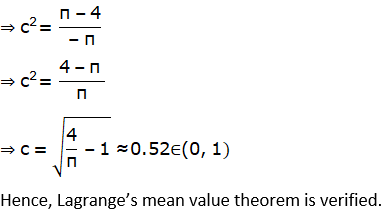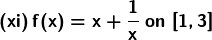Solution: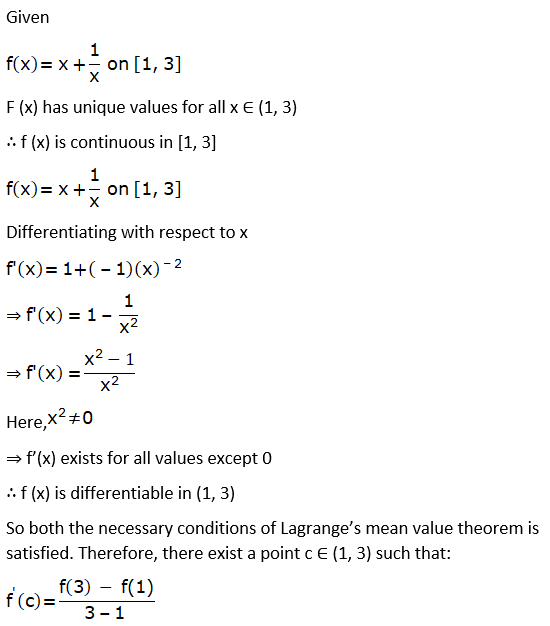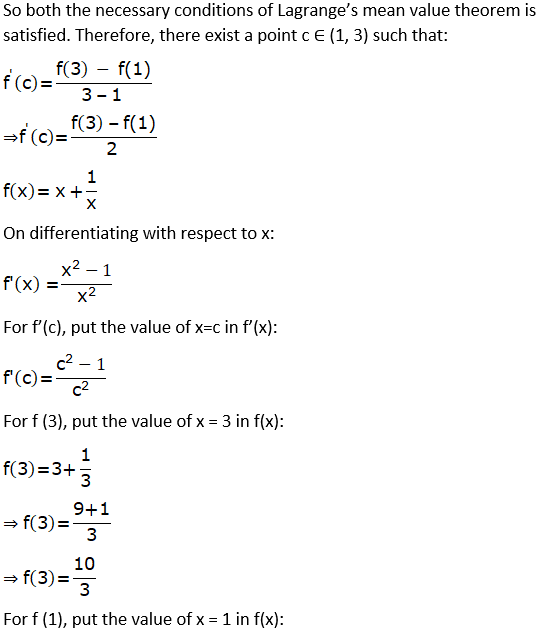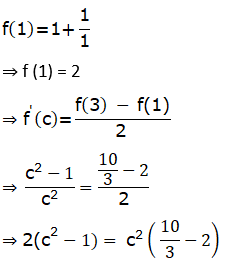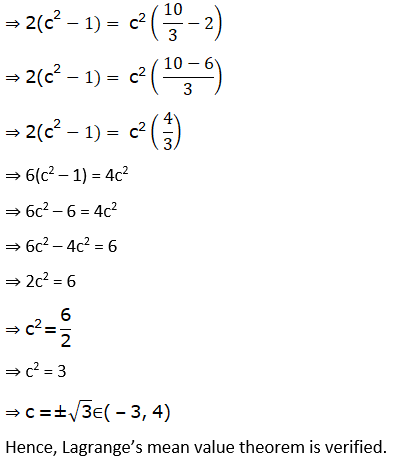(xii) f (x) = x (x + 4)2 on [0, 4]

Solution:

Given f (x) = x (x + 4)2 on [0, 4]

= x [(x)2 + 2 (4) (x) + (4)2]

= x (x2 + 8x + 16)

= x3 + 8x2 + 16x on [0, 4]

Every polynomial function is continuous everywhere on (−∞, ∞) and differentiable for all arguments. Here, f(x) is a polynomial function. So it is continuous in [0, 4] and differentiable in (0, 4). So both the necessary conditions of Lagrange’s mean value theorem is satisfied. Therefore, there exist a point c ∈ (0, 4) such that: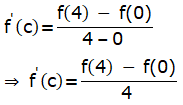f (x) = x3 + 8x2 + 16x

Differentiating with respect to x:

f’(x) = 3x2 + 8(2x) + 16

= 3x2 + 16x + 16

For f’(c), put the value of x = c in f’(x):

f’(c)= 3c2 + 16c + 16

For f (4), put the value of x = 4 in f(x):

f (4)= (4)3 + 8(4)2 + 16(4)

= 64 + 128 + 64

= 256

For f (0), put the value of x = 0 in f(x):

f (0)= (0)3 + 8(0)2 + 16(0)

= 0 + 0 + 0

= 0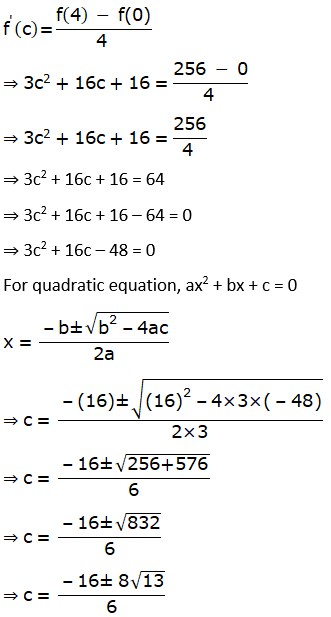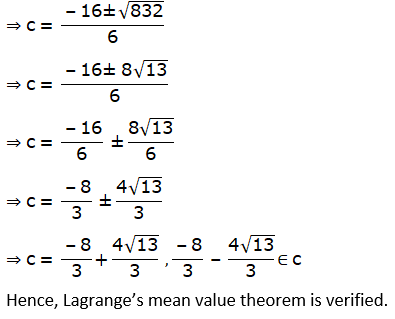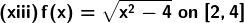Solution: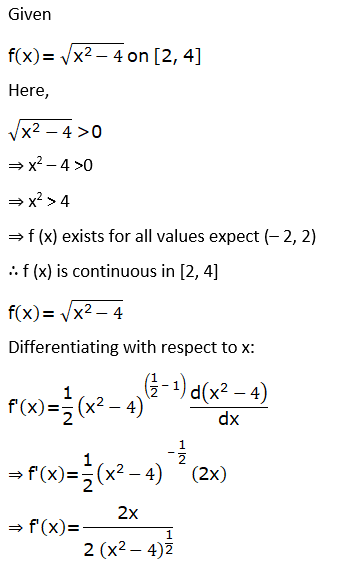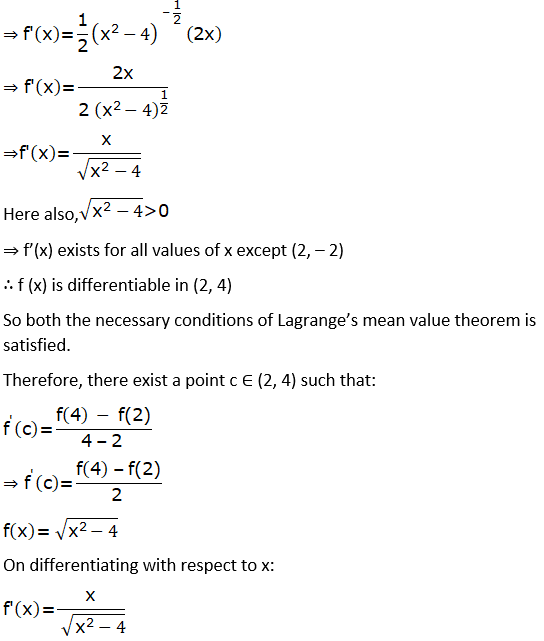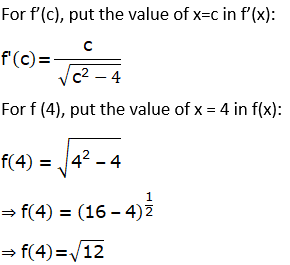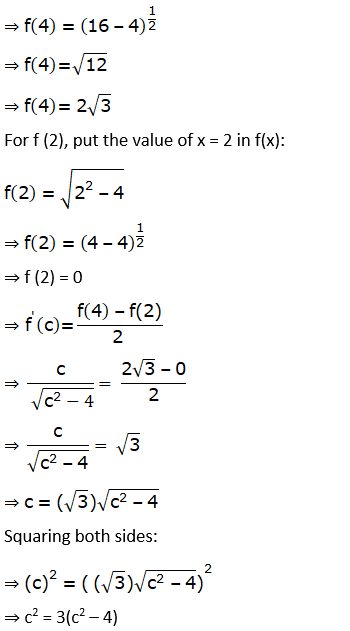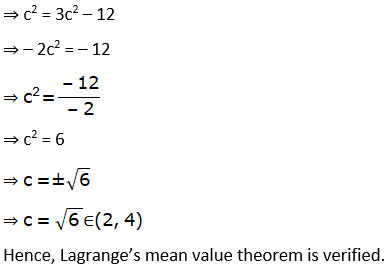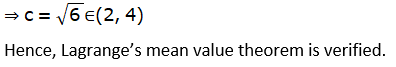(xiv) f (x) = x2 + x – 1 on [0, 4]

Solution:

Given f (x) = x2 + x – 1 on [0, 4]

Every polynomial function is continuous everywhere on (−∞, ∞) and differentiable for all arguments. Here, f(x) is a polynomial function. So it is continuous in [0, 4] and differentiable in (0, 4). So both the necessary conditions of Lagrange’s mean value theorem is satisfied. Therefore, there exist a point c ∈ (0, 4) such that: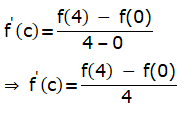f (x) = x2 + x – 1

Differentiating with respect to x:

f’(x) = 2x + 1

For f’(c), put the value of x = c in f’(x):

f’(c) = 2c + 1

For f (4), put the value of x = 4 in f(x):

f (4)= (4)2 + 4 – 1

= 16 + 4 – 1

= 19

For f (0), put the value of x = 0 in f(x):

f (0) = (0)2 + 0 – 1

= 0 + 0 – 1

= – 1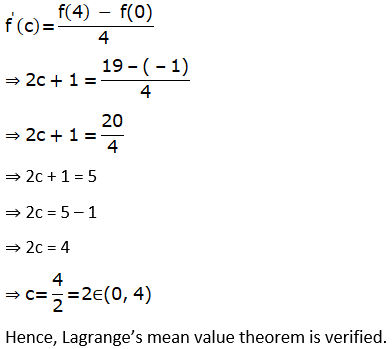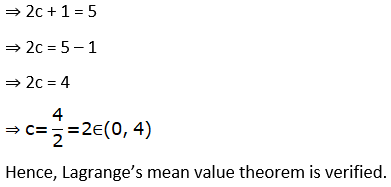(xv) f (x) = sin x – sin 2x – x on [0, π]

Solution:

Given f (x) = sin x – sin 2x – x on [0, π]

Sin x and cos x functions are continuous everywhere on (−∞, ∞) and differentiable for all arguments. So both the necessary conditions of Lagrange’s mean value theorem is satisfied. Therefore, there exist a point c ∈ (0, π) such that: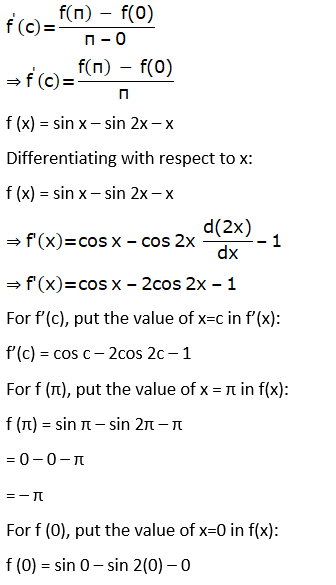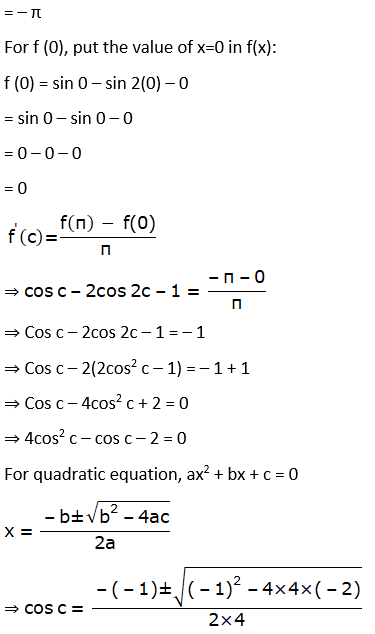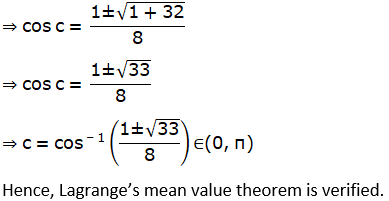(xvi) f (x) = x3 – 5x2 – 3x on [1, 3]

Solution:

Given f (x) = x3 – 5x2 – 3x on [1, 3]

Every polynomial function is continuous everywhere on (−∞, ∞) and differentiable for all arguments. Here, f(x) is a polynomial function. So it is continuous in [1, 3] and differentiable in (1, 3). So both the necessary conditions of Lagrange’s mean value theorem is satisfied.

Therefore, there exist a point c ∈ (1, 3) such that: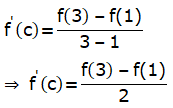f (x) = x3 – 5x2 – 3x

Differentiating with respect to x:

f’(x) = 3x2 – 5(2x) – 3

= 3x2 – 10x – 3

For f’(c), put the value of x=c in f’(x):

f’(c)= 3c2 – 10c – 3

For f (3), put the value of x = 3 in f(x):

f (3)= (3)3 – 5(3)2 – 3(3)

= 27 – 45 – 9

= – 27

For f (1), put the value of x = 1 in f(x):

f (1)= (1)3 – 5 (1)2 – 3 (1)

= 1 – 5 – 3

= – 7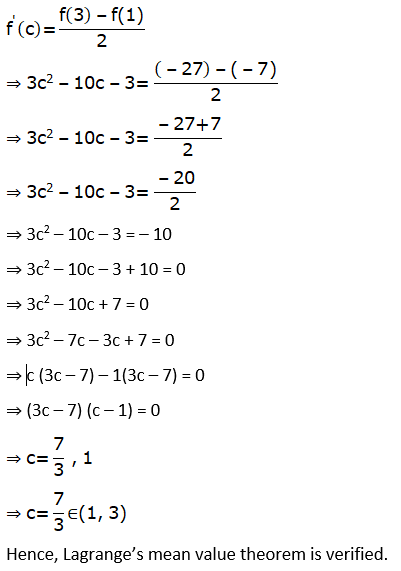⇒ 3c2 – 10c – 3 = – 10

⇒ 3c2 – 10c – 3 + 10 = 0

⇒ 3c2 – 10c + 7 = 0

⇒ 3c2 – 7c – 3c + 7 = 0

⇒ c (3c – 7) – 1(3c – 7) = 0

⇒ (3c – 7) (c – 1) = 0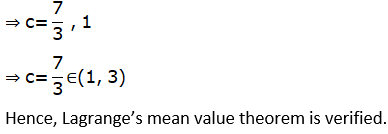2. Discuss the applicability of Lagrange’s mean value theorem for the function f(x) = |x| on [– 1, 1].

Solution: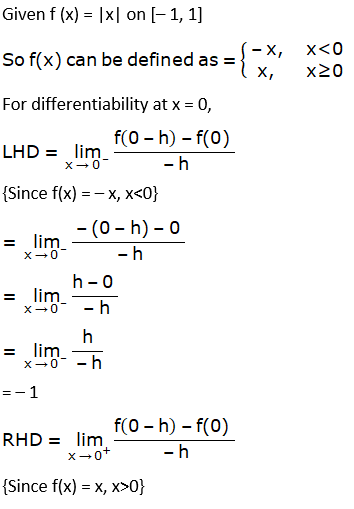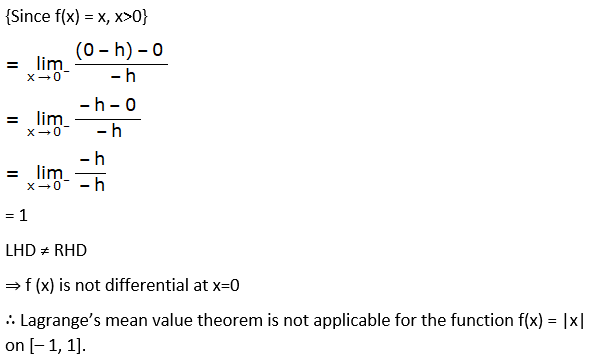3. Show that the Lagrange’s mean value theorem is not applicable to the function f(x) = 1/x on [–1, 1].

Solution:

Given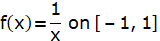Here, x ≠ 0

⇒ f (x) exists for all values of x except 0

⇒ f (x) is discontinuous at x=0

∴ f (x) is not continuous in [– 1, 1]

Hence the Lagrange’s mean value theorem is not applicable to the function f (x) = 1/x on [-1, 1]

4. Verify the hypothesis and conclusion of Lagrange’s mean value theorem for the function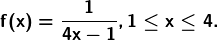Solution:

Given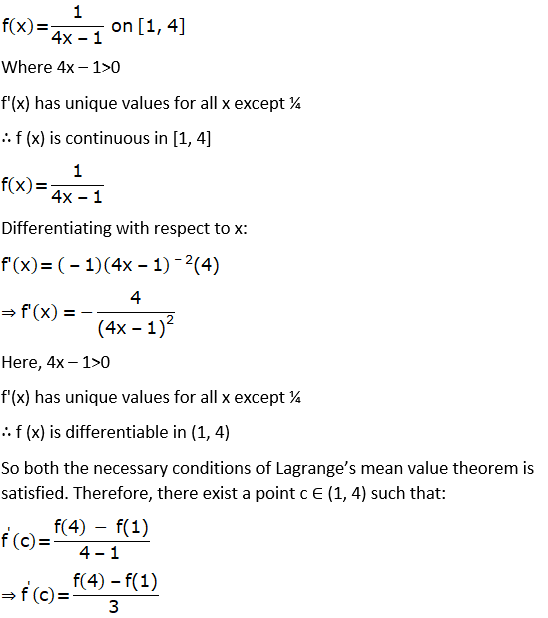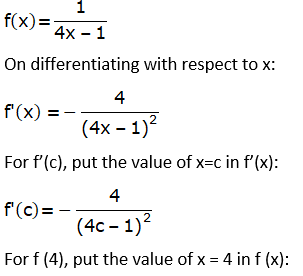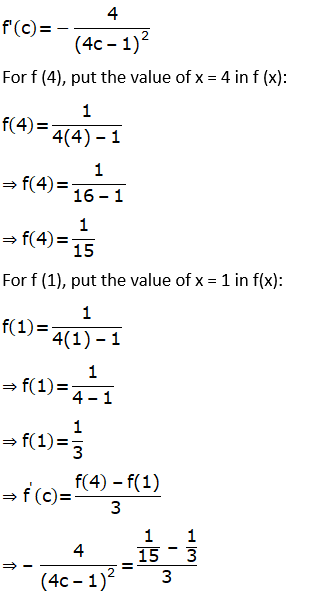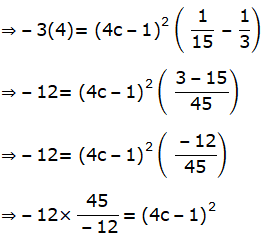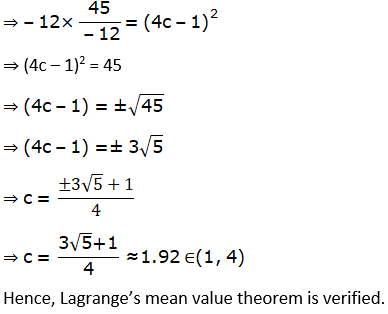5. Find a point on the parabola y = (x – 4)2, where the tangent is parallel to the chord joining (4, 0) and (5, 1).

Solution:

Given f(x) = (x – 4)2 on [4, 5]

This interval [a, b] is obtained by x – coordinates of the points of the chord.

Every polynomial function is continuous everywhere on (−∞, ∞) and differentiable for all arguments. Here, f(x) is a polynomial function. So it is continuous in [4, 5] and differentiable in (4, 5). So both the necessary conditions of Lagrange’s mean value theorem is satisfied.

Therefore, there exist a point c ∈ (4, 5) such that: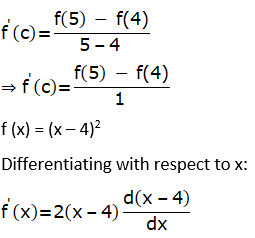⇒ f’(x) = 2 (x – 4) (1)

⇒ f’(x) = 2 (x – 4)

For f’(c), put the value of x=c in f’(x):

f’(c) = 2 (c – 4)

For f (5), put the value of x=5 in f(x):

f (5) = (5 – 4)2

= (1)2

= 1

For f (4), put the value of x=4 in f(x):

f (4) = (4 – 4)2

= (0)2

= 0

f’(c) = f(5) – f(4)

⇒ 2(c – 4) = 1 – 0

⇒ 2c – 8 = 1

⇒ 2c = 1 + 8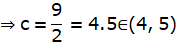We know that, the value of c obtained in Lagrange’s Mean value Theorem is nothing but the value of x – coordinate of the point of the contact of the tangent to the curve which is parallel to the chord joining the points (4, 0) and (5, 1).

Now, put this value of x in f(x) to obtain y:

y = (x – 4)2